# JP5133471B2 - Resistance variable nonvolatile element writing method and memory device - Google Patents

## Info

Publication number
JP5133471B2
JP5133471B2 JP2012538519A JP2012538519A JP5133471B2 JP 5133471 B2 JP5133471 B2 JP 5133471B2 JP 2012538519 A JP2012538519 A JP 2012538519A JP 2012538519 A JP2012538519 A JP 2012538519A JP 5133471 B2 JP5133471 B2 JP 5133471B2
Authority
JP
Japan
Prior art keywords
resistance
voltage pulse
resistance state
nonvolatile memory
electrode
Prior art date
Legal status (The legal status is an assumption and is not a legal conclusion. Google has not performed a legal analysis and makes no representation as to the accuracy of the status listed.)
Active
Application number
JP2012538519A
Other languages
Japanese (ja)
Other versions
JPWO2012132341A1 (en
Inventor

Original Assignee
パナソニック株式会社
Priority date (The priority date is an assumption and is not a legal conclusion. Google has not performed a legal analysis and makes no representation as to the accuracy of the date listed.)
Filing date
Publication date
Priority to JP2011068556 priority Critical
Priority to JP2011068556 priority
Application filed by パナソニック株式会社 filed Critical パナソニック株式会社
Priority to JP2012538519A priority patent/JP5133471B2/en
Priority to PCT/JP2012/001975 priority patent/WO2012132341A1/en
Application granted granted Critical
Publication of JP5133471B2 publication Critical patent/JP5133471B2/en
Publication of JPWO2012132341A1 publication Critical patent/JPWO2012132341A1/en
Application status is Active legal-status Critical
Anticipated expiration legal-status Critical

## Images

•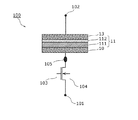••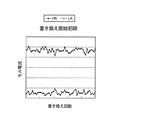•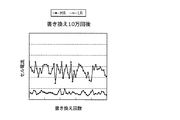•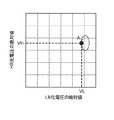•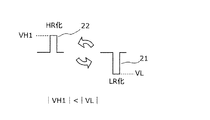•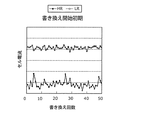•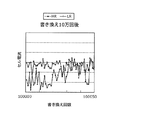•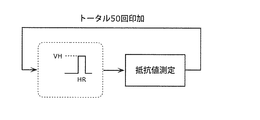•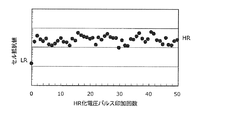•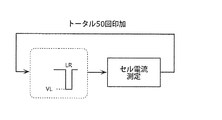•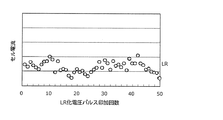•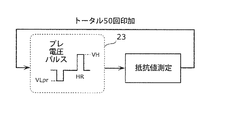••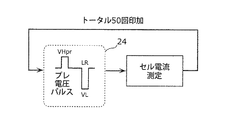•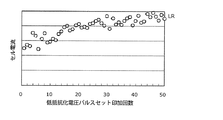•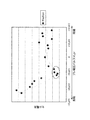•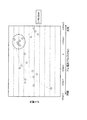•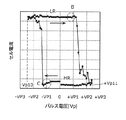•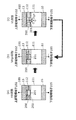•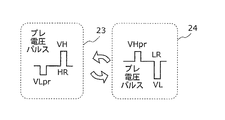•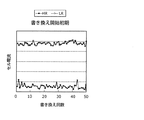•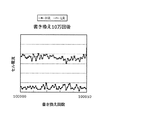•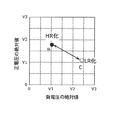••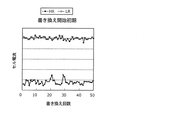•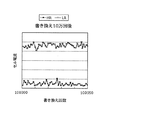•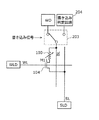•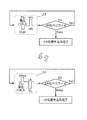•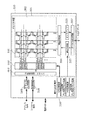•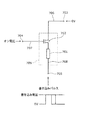••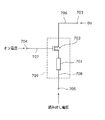•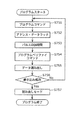•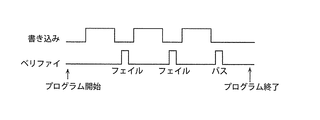## Classifications

• GPHYSICS
• G11INFORMATION STORAGE
• G11CSTATIC STORES
• G11C13/00Digital stores characterised by the use of storage elements not covered by groups G11C11/00, G11C23/00 - G11C25/00
• G11C13/0002Digital stores characterised by the use of storage elements not covered by groups G11C11/00, G11C23/00 - G11C25/00 using resistive RAM [RRAM] elements
• G11C13/0021Auxiliary circuits
• G11C13/0069Writing or programming circuits or methods
• GPHYSICS
• G11INFORMATION STORAGE
• G11CSTATIC STORES
• G11C13/00Digital stores characterised by the use of storage elements not covered by groups G11C11/00, G11C23/00 - G11C25/00
• G11C13/0002Digital stores characterised by the use of storage elements not covered by groups G11C11/00, G11C23/00 - G11C25/00 using resistive RAM [RRAM] elements
• G11C13/0007Digital stores characterised by the use of storage elements not covered by groups G11C11/00, G11C23/00 - G11C25/00 using resistive RAM [RRAM] elements comprising metal oxide memory material, e.g. perovskites
• GPHYSICS
• G11INFORMATION STORAGE
• G11CSTATIC STORES
• G11C13/00Digital stores characterised by the use of storage elements not covered by groups G11C11/00, G11C23/00 - G11C25/00
• G11C13/0002Digital stores characterised by the use of storage elements not covered by groups G11C11/00, G11C23/00 - G11C25/00 using resistive RAM [RRAM] elements
• G11C13/0021Auxiliary circuits
• G11C13/0064Verifying circuits or methods
• GPHYSICS
• G11INFORMATION STORAGE
• G11CSTATIC STORES
• G11C13/00Digital stores characterised by the use of storage elements not covered by groups G11C11/00, G11C23/00 - G11C25/00
• G11C13/0002Digital stores characterised by the use of storage elements not covered by groups G11C11/00, G11C23/00 - G11C25/00 using resistive RAM [RRAM] elements
• G11C13/0021Auxiliary circuits
• G11C13/0069Writing or programming circuits or methods
• G11C2013/0073Write using bi-directional cell biasing
• GPHYSICS
• G11INFORMATION STORAGE
• G11CSTATIC STORES
• G11C13/00Digital stores characterised by the use of storage elements not covered by groups G11C11/00, G11C23/00 - G11C25/00
• G11C13/0002Digital stores characterised by the use of storage elements not covered by groups G11C11/00, G11C23/00 - G11C25/00 using resistive RAM [RRAM] elements
• G11C13/0021Auxiliary circuits
• G11C13/0069Writing or programming circuits or methods
• G11C2013/0083Write to perform initialising, forming process, electro forming or conditioning
• GPHYSICS
• G11INFORMATION STORAGE
• G11CSTATIC STORES
• G11C13/00Digital stores characterised by the use of storage elements not covered by groups G11C11/00, G11C23/00 - G11C25/00
• G11C13/0002Digital stores characterised by the use of storage elements not covered by groups G11C11/00, G11C23/00 - G11C25/00 using resistive RAM [RRAM] elements
• G11C13/0021Auxiliary circuits
• G11C13/0069Writing or programming circuits or methods
• G11C2013/0092Write characterized by the shape, e.g. form, length, amplitude of the write pulse

## Description

The present invention relates to a resistance change nonvolatile memory element writing method and a resistance change nonvolatile memory device, and more particularly, resistance change of a resistance change nonvolatile memory element whose resistance value reversibly changes based on an electrical signal. TECHNICAL FIELD The present invention relates to a writing method and a resistance change type nonvolatile memory device for stably maintaining memory.

Nonvolatile storage devices are widely installed in portable devices such as mobile phones and digital cameras, and their use is rapidly expanding. In recent years, opportunities for handling audio data and image data have increased, and there is a strong demand for a nonvolatile memory device that has a larger capacity and operates at a higher speed. In the field of non-volatile storage devices for portable devices, there is an increasing demand for low power consumption.

The mainstream of current nonvolatile storage devices is flash memory. The flash memory stores data by controlling charges accumulated in the floating gate. The flash memory has a structure in which electric charges are stored in a floating gate in a high electric field, so that there is a limit to miniaturization, and there is a problem that it is difficult to perform fine processing necessary for further increase in capacity. Further, in a flash memory, it is necessary to erase a predetermined block at once for rewriting. Due to such characteristics, it takes a very long time to rewrite the flash memory, and there is a limit to random access and high speed.

As a next-generation nonvolatile memory device that solves these problems, there is one using a resistance variable element that records information by a change in electrical resistance. Non-volatile semiconductor devices (also referred to as “non-volatile memories”) that use resistance-change elements that are currently proposed include MRAM (Magnetic RAM), PCRAM (Phase-Change RAM), ReRAM (Resistive RAM), and the like. Has been proposed (see, for example, Patent Documents 1 to 3).

JP 2004-185756 A US Pat. No. 5,287,317 JP 2004-234707 A

However, in a nonvolatile memory device using a conventional variable resistance element using, for example, a bipolar ReRAM, a problem in writing occurs when a verify operation and an additional writing associated therewith are performed.

Accordingly, the present invention has been made in view of the above-described circumstances, and an object thereof is to provide a resistance change type nonvolatile memory element writing method in which the stability and reliability of the writing operation are improved.

In order to achieve the above object, a method of writing a variable resistance nonvolatile memory element according to an aspect of the present invention includes applying a voltage pulse to a memory cell including a variable resistance nonvolatile memory element. A resistance change type nonvolatile memory element is a writing method in which a first resistance state and a second resistance state are reversibly changed according to the polarity of an applied voltage pulse, wherein the resistance change type nonvolatile memory element includes: , A first electrode, a second electrode, and a resistance change layer sandwiched between the first electrode and the second electrode, the resistance change layer being an oxygen-deficient first in contact with the first electrode. And the second transition metal oxide layer in contact with the second electrode and having a lower oxygen deficiency than the first transition metal oxide layer, the resistance variable nonvolatile memory The memory element includes the first electrode and When a first voltage pulse that is a voltage pulse equal to or higher than a first threshold voltage having a positive potential with respect to the other of the first electrode and the second electrode with respect to one of the second electrodes is applied, Transition to a first resistance state, which is equal to or higher than a second threshold voltage having a positive potential with respect to the one of the first electrode and the second electrode with respect to the other of the first electrode and the second electrode When the second voltage pulse that is the voltage pulse is applied, the write method has a characteristic of transitioning to the second resistance state, and the writing method is characterized in that the variable resistance nonvolatile memory element is moved from the second resistance state to the second resistance state. When changing to the first resistance state, the absolute value of the voltage is smaller than that of the second threshold voltage and the polarity of the first voltage pulse is different from that of the first voltage pulse. Mark 1 resistance pre-voltage pulse A first step of, after said first step includes a first resistance state of the step and a second step of applying the first voltage pulse.

ADVANTAGE OF THE INVENTION According to this invention, the write-in method of the resistance change non-volatile memory element which improved the stability and reliability of write-in operation | movement is realizable.

FIG. 1 is a schematic diagram showing a configuration of a 1T1R type memory cell using a resistance change element according to the present invention. FIG. 2A is a schematic diagram of a pulse waveform in the case where application of a high resistance (HR) voltage pulse (one pulse) and application of a low resistance (LR) voltage pulse (1 pulse) are alternately performed. FIG. 2B is a rewrite characteristic diagram at the beginning of rewrite when the high resistance voltage pulse and the low resistance voltage pulse shown in FIG. 2A are alternately applied. FIG. 2C is a rewrite characteristic diagram after 100,000 rewrites when the high resistance voltage pulse and the low resistance voltage pulse shown in FIG. 2A are alternately applied. FIG. 3 is a diagram showing the operating point of the rewrite voltage when the high resistance (HR) voltage pulse and the low resistance (LR) voltage pulse shown in FIG. 2A are repeatedly applied alternately. FIG. 4A is a schematic diagram of another pulse waveform when the application of the high resistance (HR) voltage pulse (one pulse) and the application of the low resistance (LR) voltage pulse (1 pulse) are alternately performed. . FIG. 4B is a rewrite characteristic diagram at the beginning of rewrite when the high resistance voltage pulse and the low resistance voltage pulse shown in FIG. 4A are alternately applied. FIG. 4C is a rewrite characteristic diagram after 100,000 rewrites when the high resistance voltage pulse and the low resistance voltage pulse shown in FIG. 4A are alternately applied. FIG. 5A is a diagram showing a resistance value measurement flow when a high resistance (HR) voltage pulse is continuously applied in the present invention. FIG. 5B is a resistance change characteristic diagram measured based on the measurement flow shown in FIG. 5A. FIG. 6A is a diagram showing a cell current measurement flow when applying a low resistance (LR) voltage pulse sequence in the present invention. FIG. 6B is a cell current change characteristic diagram measured based on the measurement flow shown in FIG. 6A. FIG. 7A is a diagram showing a resistance value measurement flow in the case where high resistance voltage pulse set application is continuously performed in the present invention. FIG. 7B is a resistance change characteristic diagram measured based on the resistance value measurement flow shown in FIG. 7A. FIG. 8A is a diagram showing a cell current measurement flow in the case where low resistance voltage pulse set application is continuously performed in the present invention. FIG. 8B is a cell current change characteristic diagram measured based on the cell current measurement flow shown in FIG. 8A. FIG. 9 is a characteristic diagram showing the dependence of the HR cell current on the pre-voltage pulse in the present invention. FIG. 10 is a characteristic diagram showing the dependence of the LR cell current on the pre-voltage pulse in the present invention. FIG. 11 is a diagram showing the pulse VI characteristics of the memory cell of the present invention. FIG. 12 is a diagram for explaining an estimation mechanism at the time of LR writing by applying a pre-voltage pulse according to the present invention. FIG. 13A is a schematic diagram of a pulse waveform when the high resistance (HR) voltage pulse set application and the low resistance (LR) voltage pulse set application are alternately performed in the first embodiment. FIG. 13B is a rewrite characteristic diagram at the beginning of rewriting when the application of the high resistance voltage pulse set and the application of the low resistance voltage pulse set shown in FIG. 13A are alternately performed. FIG. 13C is a rewrite characteristic diagram after 100,000 rewrites when the high resistance voltage pulse set application and the low resistance voltage pulse set application shown in FIG. 13A are alternately performed. FIG. 14 is a diagram for explaining the operating point of the rewrite voltage when the high resistance (HR) voltage pulse set and the low resistance (LR) voltage pulse set shown in FIG. 13A are repeatedly applied alternately. . FIG. 15A is a schematic diagram of a pulse waveform when the high resistance (HR) voltage pulse set application is continuously performed M times and the low resistance (LR) voltage pulse set application is alternately performed N times in the second embodiment. FIG. FIG. 15B is a rewriting characteristic diagram at the beginning of rewriting when continuous application of the high resistance voltage pulse set and continuous application of the low resistance voltage pulse set shown in FIG. 15A are alternately performed. FIG. 15C is a rewrite characteristic diagram after 100,000 rewrites when the continuous application of the high resistance voltage pulse set and the continuous application of the low resistance voltage pulse set shown in FIG. 15A are alternately performed. FIG. 16 is an example of an equivalent circuit of a circuit configuration of a portion necessary for rewriting in the present invention. FIG. 17 is a diagram for explaining an operation flow of verify writing in the present invention. FIG. 18 is a block diagram showing a configuration of a nonvolatile memory device having memory cells having a specific array structure in the present invention. FIG. 19 is a diagram showing a voltage pulse application state when a write operation is performed in the memory cell of Patent Document 1 in the prior art. FIG. 20 is a diagram showing a voltage pulse application state when an erase operation is performed in the memory cell of Patent Document 1 in the prior art. FIG. 21 is a diagram showing a voltage pulse application state when a read operation is performed in the memory cell of Patent Document 1 in the prior art. FIG. 22 is a flowchart of the conventional verify operation shown in Patent Documents 2 and 3 in the prior art. FIG. 23 is a timing chart showing the flow of the conventional verify operation shown in Patent Documents 2 and 3 in the prior art.

(Background to obtaining one embodiment of the present invention)
The present inventor has found that the following problems occur with respect to the conventional nonvolatile memory device described in the “Background Art” section. The problem will be described below.

Patent Document 1 discloses an example of a control method of a bipolar ReRAM element using an oxide having a perovskite structure. Here, the bipolar type means that a voltage pulse having a different polarity causes the ReRAM element to change to a high resistance state with a voltage pulse of one polarity, and changes to a low resistance state with a voltage pulse of the other polarity. The ReRAM element is at least a low resistance state (also referred to as “LR state” or simply “LR”) by electrical stimulation, and a high resistance state (“HR state” or simply “HR”) having a higher resistance value than the low resistance state. An element that can be reversibly changed. A nonvolatile semiconductor device that stores information according to a low resistance state or a high resistance state.

Hereinafter, a method for controlling the ReRAM element will be described with reference to the drawings.

19 to 21 are diagrams showing a configuration of the memory cell 709 disclosed in Patent Document 1 and a control method thereof. The memory cell 709 includes a resistance variable element 701 and a selection transistor 702. One terminal of the resistance variable element 701 and one main terminal (drain or source) of the selection transistor 702 are electrically connected to each other. The other main terminal (source or drain) of the selection transistor 702 is electrically connected to the source line terminal 703 by the source line 706. The other terminal of the resistance variable element 701 is electrically connected to the bit line terminal 705 by the bit line 708. The gate of the selection transistor 702 is electrically connected to the word line terminal 704 through a word line 707. When writing data (when writing “1” (where data “1” is assigned to the HR state of the ReRAM element)), when erasing (when writing “0” (where data “0” is In both the case of the ReRAM element LR) and the case of reading, a high level on-voltage is applied to the word line terminal 704 of the selected memory cell, and the selection transistor 702 is turned on.

FIG. 19 is a diagram illustrating a voltage pulse application state when performing a write operation in the memory cell 709 of Patent Document 1. In FIG. The source line 706 is set to 0 V (grounded), a positive write pulse having a predetermined write voltage amplitude is applied to the bit line 708, and desired data is written to the resistance variable element 701. When multi-value information is written to the resistance variable element 701, the voltage amplitude of the write pulse is set to a level corresponding to the value of the data to be written. For example, when quaternary data is written to one resistance variable element 701, one of four predetermined voltage amplitudes determined corresponding to each value of the write data is selected and the write operation is performed. Is called. As the write pulse width, an appropriate width corresponding to the element is selected. That is, in order to change to a predetermined resistance state, there is one voltage amplitude level and pulse width corresponding to the resistance state.

FIG. 20 is a diagram illustrating a voltage pulse application state when an erase operation is performed in the memory cell 709 of Patent Document 1. In FIG. The bit line 708 is set to 0 V (grounded), and a positive erase pulse having a predetermined erase voltage amplitude is applied to the source line 706. By applying the erase pulse, the electric resistance of the resistance variable element 701 becomes a minimum value. In Patent Document 1, when an erase pulse is applied to a specific source line 706 with a plurality of bit lines 708 set to 0 V, a plurality of memories connected to the plurality of bit lines 708 and the source line 706 are disclosed. It is disclosed that cells are erased simultaneously.

FIG. 21 is a diagram illustrating a voltage pulse application state when a read operation is performed in the memory cell 709 of Patent Document 1. In FIG. When data stored in the resistance variable element 701 is read, the source line 706 is set to 0 V (grounded), and a predetermined read voltage is applied to the selected bit line 708 via a read circuit. When the read voltage is applied, the level of the bit line 708 is compared with the reference level for reading by the comparison / determination circuit, and the stored data is read.

In Patent Document 2 and Patent Document 3, in a general semiconductor memory that can be electrically erased or written, or in a resistance change type memory such as ReRAM, an electrical state written in order to improve the reliability of write data. Has been proposed for a verify operation that verifies whether or not satisfies a desired threshold. That is, in the case of data writing, as shown in FIG. 22, after inputting a program command (for example, “write”) (S751), an address and data are input, and address / data latching (S752) is performed. Application of a program pulse to the cell is started, and data is written to the memory cell (S753). After the application of the program pulse is stopped, the program verify mode is entered by inputting a program verify command (S754), and data reading from the memory cell to which data has been written is started (S55). Reading is performed, and the read data is compared with the first input expected value data (S756). If they match (Yes in S756), the program ends normally and the reading mode is set ( S757), the program is terminated. On the other hand, if the data do not match (No in S756), the program pulse is applied again and additional writing is performed (S751 to S753). This series of operations is repeated until all data matches. However, in practice, the upper limit number of repetitions is often set to avoid an infinite loop. FIG. 23 is a timing chart showing that the program is terminated because a series of operations for executing the verify operation is performed after the program pulse is applied, and the expected value data and the written data match at the third time. That is, according to such a verify operation, the physical characteristics written in the non-volatile semiconductor device satisfy a desired level, and a sufficient margin is provided for the threshold value for determining to restore the original digital information. Can be secured, and further improvement of data reliability can be secured.

However, in a nonvolatile memory device using a conventional variable resistance element using, for example, a bipolar ReRAM, a problem in writing occurs when a verify operation and an additional writing associated therewith are performed. Hereinafter, a problem that occurs will be described.

For example, in order to improve the stability and reliability of the operation in the nonvolatile memory device using ReRAM, the resistance level written by the verify operation is checked after the ReRAM write operation and the desired resistance value is not satisfied. Assume that additional writing is performed. However, although the desired resistance value is satisfied at the time of the verify operation executed immediately after the write operation is performed, the resistance value gradually changes after a short period of time, and the threshold resistance value used for verification is reduced. A writing failure that changes to an unsatisfactory level occurs.

In general, a physical quantity written in a memory cell often fluctuates due to standing for a long time, standing at a high temperature, and deterioration of the material composition due to the number of rewritings. Based on the reliability specifications required for such fluctuations, it is required to write so that the initial physical quantity satisfies a predetermined condition. That is, when the written physical quantity is compared with the determined threshold value and decoded into the original digital data, writing is performed so that an appropriate margin can be secured between the written physical quantity and the threshold value. In order to secure such a margin, a verify operation is executed. However, immediately after the verification operation is performed and it is determined that the planned level is satisfied, if the written physical quantity changes abruptly so as to approach the threshold value, the above margin cannot be secured, and the required reliability is guaranteed. Can not. This is a fatal problem for nonvolatile memories. This ReRAM has the advantage of high speed that can be written in a short time of several tens of ns, and has the advantage of excellent reliability that long-term data can be retained even in a high temperature environment if normal writing can be performed. It has high potential as a next-generation semiconductor memory that replaces memory. However, even if there are rare bits that cannot be found defective even if the verify operation as described above is performed, the excellent performance of the ReRAM cannot be utilized as a whole device when such bits are generated.

In response to such a problem, the inventors have found that the number of bits in which a write failure occurs can be significantly improved by a unique write step.

As described above, the biggest problem of the defective phenomenon in writing is that the write resistance value fluctuates after execution of the verify operation due to insufficient write conditions, and a bit that interrupts the verify threshold level is generated. is there. Such a failure bit is randomly generated in the memory cell array, and in the verification executed immediately after data is written to the memory cell, it cannot be identified whether the data has been normally written, and the failure is missed. Originally, a predetermined margin is provided by verification in order to ensure the data reliability required for the nonvolatile semiconductor device against deterioration factors such as long-term storage, high-temperature storage, and a large number of rewrite cycles. However, when the above-described problem occurs, a necessary margin cannot be ensured by verification, and the reliability required at the time of data reading cannot be guaranteed.

In view of the above-described circumstances, the present inventor has come up with a method of writing a variable resistance nonvolatile memory element that improves the stability and reliability of the write operation. Specifically, the present inventors have come up with a resistance change nonvolatile memory element writing method that suppresses the amount of decrease in the operation window due to an increase in the number of rewrites and makes it possible to stably maintain the resistance change operation.

In order to achieve the above object, a method of writing a variable resistance nonvolatile memory element according to an aspect of the present invention includes applying a voltage pulse to a memory cell including a variable resistance nonvolatile memory element. A resistance change type nonvolatile memory element is a writing method in which a first resistance state and a second resistance state are reversibly changed according to the polarity of an applied voltage pulse, wherein the resistance change type nonvolatile memory element includes: , A first electrode, a second electrode, and a resistance change layer sandwiched between the first electrode and the second electrode, the resistance change layer being an oxygen-deficient first in contact with the first electrode. And the second transition metal oxide layer in contact with the second electrode and having a lower oxygen deficiency than the first transition metal oxide layer, the resistance variable nonvolatile memory The memory element includes the first electrode and When a first voltage pulse that is a voltage pulse equal to or higher than a first threshold voltage having a positive potential with respect to the other of the first electrode and the second electrode with respect to one of the second electrodes is applied, Transition to a first resistance state, which is equal to or higher than a second threshold voltage having a positive potential with respect to the one of the first electrode and the second electrode with respect to the other of the first electrode and the second electrode When the second voltage pulse that is the voltage pulse is applied, the write method has a characteristic of transitioning to the second resistance state, and the writing method is characterized in that the variable resistance nonvolatile memory element is moved from the second resistance state to the second resistance state. When changing to the first resistance state, the absolute value of the voltage is smaller than that of the second threshold voltage and the polarity of the first voltage pulse is different from that of the first voltage pulse. Mark 1 resistance pre-voltage pulse A first step of, after said first step includes a first resistance state of the step and a second step of applying the first voltage pulse.

This makes it possible to improve the high resistance capability and the low resistance capability by applying in advance appropriate pre-voltage pulses that have different polarities from the high-resistance voltage pulse and the low-resistance voltage pulse. Even if this increases, an appropriate operation window can be secured, and the reliability of the nonvolatile memory device can be improved. Accordingly, it is possible to realize a variable resistance nonvolatile memory element writing method that suppresses a decrease in the operation window due to endurance (increase in the number of rewrites) and stably maintains the resistance change operation.

Here, in the writing method, even if the variable resistance nonvolatile memory element is changed from the second resistance state to the first resistance state by repeating the first step and the second step. Good.

The first resistance state may be a high resistance state, the second resistance state may be a low resistance state having a resistance lower than that of the high resistance state, and the first resistance state may be a low resistance state. The second resistance state may be a high resistance state in which the resistance is higher than the resistance in the low resistance state.

Further, in the writing method, it is further determined whether or not the first resistance state writing for changing the variable resistance nonvolatile memory element to the first resistance state by the first resistance state step is completed. Including a first resistance state determination step, wherein the first resistance state determination step is performed after the first resistance state step, and the first resistance state step and the first resistance state step The resistance state determination step may be repeated until the resistance state of the variable resistance nonvolatile memory element reaches the predetermined first resistance state.

As a result, by setting the number of continuous application of the resistance change voltage pulse set, whichever is weaker, HR capability or LR capability, the balance between HR capability and LR capability is appropriate without increasing the write voltage. As a result, an appropriate operation window can be secured even if the number of rewrites is increased, and the reliability of the nonvolatile memory device can be greatly improved and a low voltage operation is also possible.

Further, the writing method further includes the step of changing the resistance variable nonvolatile memory element with respect to the resistance variable nonvolatile memory element when the resistance variable nonvolatile memory element is changed from the first resistance state to the second resistance state. A third step of applying a second resistance pre-voltage pulse having a voltage absolute value smaller than the threshold voltage of 1 and having a polarity different from that of the second voltage pulse, and after the third step, A second resistance state setting step including a fourth step of applying two voltage pulses may be included.

Here, in the writing method, even if the variable resistance nonvolatile memory element is changed from the first resistance state to the second resistance state by repeating the third step and the fourth step. Good.

The writing method may further include a first variable state in which the variable resistance nonvolatile memory element is changed to the first resistance state by applying the first voltage pulse in the first resistance state setting step. In the first resistance state determination step for determining whether or not the resistance state writing is completed, and in the second resistance state step, the second voltage pulse is applied to thereby change the resistance variable nonvolatile memory. A second resistance state determination step for determining whether or not the second resistance state write for changing the volatile memory element to the second resistance state is completed, and including the first resistance state determination step The step is performed after the first resistance state setting step, and the second resistance state determination step is performed after the second resistance state step, and the first resistance state And the first resistance state determination step are repeated until the resistance state of the variable resistance nonvolatile memory element reaches a predetermined first resistance state, and the second resistance state step And the second resistance state determination step may be repeated until the resistance state of the variable resistance nonvolatile memory element reaches the predetermined second resistance state.

The transition metal constituting the first transition metal oxide layer and the second transition metal oxide layer may be composed of any one of tantalum, hafnium, and zirconium.

In addition, the first transition metal constituting the first transition metal oxide layer and the second transition metal constituting the second transition metal oxide layer are different from each other, and the second transition metal standard electrode The potential may be lower than the standard electrode potential of the second transition metal.

The first transition metal oxide layer is a layer having a composition represented by TaO x (0.8 ≦ x ≦ 1.9), and the second transition metal oxide layer is TaO y. (However, it may be a layer having a composition represented by x <y).

To achieve the above object, a variable resistance nonvolatile memory device according to one embodiment of the present invention applies a voltage pulse to a memory cell including a variable resistance nonvolatile memory element and the memory cell. Thus, the variable resistance nonvolatile memory element includes a write control unit that performs writing to reversibly change the first resistance state and the second resistance state according to the polarity of the applied voltage pulse, and The variable resistance nonvolatile memory element includes a first electrode, a second electrode, and a variable resistance layer sandwiched between the first electrode and the second electrode, and the variable resistance layer includes the first electrode. An oxygen-deficient first transition metal oxide layer in contact with the second electrode, and a second transition metal oxide layer in contact with the second electrode and having a lower oxygen deficiency than the first transition metal oxide layer. Including the variable resistance nonvolatile memory The child is a voltage pulse equal to or higher than a first threshold voltage having a positive potential with respect to the other of the first electrode and the second electrode with respect to one of the first electrode and the second electrode. When a voltage pulse of 1 is applied, the state transitions to the first resistance state and is positive with respect to the one of the first electrode and the second electrode with respect to the other of the first electrode and the second electrode. When the second voltage pulse, which is a voltage pulse having a potential equal to or higher than the second threshold voltage, is applied, the write control unit has a characteristic of transitioning to the second resistance state. When the memory element is changed from the second resistance state to the first resistance state, the absolute value of the voltage is smaller than the second threshold voltage with respect to the resistance variable nonvolatile memory element, and The polarity of the first voltage pulse is A first step of applying a first resistance pre voltage pulse consisting, then executes the processing of the first resistance state of the step and a second step of applying the first voltage pulse.

Here, the memory cell may be a variable resistance nonvolatile memory device in which a variable resistance nonvolatile memory element and a switch element are connected in series.

ADVANTAGE OF THE INVENTION According to this invention, the write-in method of the resistance change non-volatile memory element which improved the stability and reliability of write-in operation | movement is realizable. That is, it is possible to realize a resistance change type nonvolatile memory element writing method that suppresses a decrease in the operation window due to an increase in the number of rewrites and makes it possible to stably maintain the resistance change operation.

More specifically, according to the variable resistance nonvolatile memory element writing method of the present invention, an appropriate pre-voltage pulse having a polarity different from that of the high-resistance voltage pulse or the low-resistance voltage pulse, By applying the voltage before applying the low resistance voltage pulse, it is possible to improve the high resistance capability and the low resistance capability. As a result, even if the number of rewrites increases, it is possible to ensure an appropriate operation window, and non-volatile The reliability of the storage device can be improved. Furthermore, by setting the number of continuous application of the resistance change voltage pulse set, whichever is weaker, which is either high resistance (HR) capability or low resistance (LR) capability, HR can be achieved without increasing the write voltage. As a result, it is possible to ensure an appropriate operation window even if the number of rewrites is increased, and to greatly improve the reliability of the nonvolatile memory device and to operate at a low voltage. Is also possible.

Note that such a resistance change type nonvolatile memory element writing method and resistance change type nonvolatile memory device include a semiconductor integrated circuit (LSI) having a part or all of the functions of such a resistance change type nonvolatile memory element. It can be applied as

Hereinafter, embodiments of the present invention will be described in detail with reference to the drawings. Each of the embodiments described below shows a preferred specific example of the present invention. The numerical values, shapes, materials, constituent elements, arrangement positions and connecting forms of the constituent elements, steps, order of steps, and the like shown in the following embodiments are merely examples, and are not intended to limit the present invention. Among the constituent elements in the following embodiments, constituent elements that are not described in the independent claims showing the highest concept of the present invention will be described as constituent elements constituting a more preferable form.

As one of variable resistance nonvolatile memory devices, a variable resistance element and a switch using tantalum (Ta), which is one of transition metals, and composed of a variable resistance layer of an oxygen-deficient oxide (tantalum oxide) A variable resistance nonvolatile memory device in which a memory cell is configured with elements will be described below. Here, the oxygen-deficient oxide refers to an oxide whose oxygen content is insufficient with respect to the stoichiometric composition. Also, in the following, as a variable resistance nonvolatile memory device using a variable resistance element, a MOS transistor and a variable resistance element are connected in series near the intersection of a bit line and a word line that are arranged orthogonal to each other. An example of a nonvolatile memory device in which memory cells called 1T1R type connected to 1 are arranged in a matrix form will be described. In the 1T1R type memory cell, one end of the two-terminal resistance change element is connected to the bit line or the source line, and the other end is connected to the drain or source of the transistor. The gate of the transistor is connected to the word line. The other end of the transistor is connected to a source line or a bit line to which one end of the variable resistance element is not connected. The source line is arranged in parallel with the bit line or the word line. Note that the configuration of the memory cell is not limited to the 1T1R type configuration. For example, as another memory cell configuration, a so-called 1D1R type cross-point memory cell in which a diode and a resistance change element are connected in series at the intersection of a bit line and a word line arranged orthogonal to each other Good.

(Embodiment 1)
FIG. 1 is a schematic diagram showing a configuration (configuration corresponding to one bit) of a 1T1R type memory cell using a resistance change element according to the present invention. This 1T1R type memory cell is a memory cell in which a variable resistance nonvolatile memory element and a switch element are connected in series. Specifically, as shown in FIG. It consists of and. The transistor 104 normally uses an NMOS transistor, but a PMOS transistor may be used.

The variable resistance element 100 is a variable resistance nonvolatile memory element according to the present invention, and is sandwiched between a first electrode (lower electrode 10), a second electrode (upper electrode 13), a first electrode, and a second electrode. The resistance change layer 11 includes an oxygen-deficient first transition metal oxide layer 111 in contact with the first electrode (lower electrode 10), and a second electrode (upper electrode 13). The second transition metal oxide layer 112 having a smaller oxygen deficiency than the first transition metal oxide layer 111 is in contact therewith. Here, the degree of oxygen deficiency refers to the ratio of oxygen deficiency with respect to the amount of oxygen constituting the oxide of the stoichiometric composition in each transition metal. For example, when the transition metal is tantalum (Ta), the stoichiometric oxide composition is Ta 2 O 5 , and thus can be expressed as TaO 2.5 . The degree of oxygen deficiency of TaO 2.5 is 0%. For example, the oxygen deficiency of an oxygen deficient tantalum oxide having a composition of TaO 1.5 is oxygen deficiency = (2.5−1.5) /2.5=40%.

Specifically, as illustrated in FIG. 1, the resistance change element 100 includes a first electrode (lower electrode 10) and a low-resistance first transition metal oxide layer including an oxygen-deficient tantalum oxide. 111 (TaO x , 0 <x <2.5) and a high-resistance second transition metal oxide layer 112 (TaO y , x <y) are stacked, and the second electrode (upper electrode 13 ) And are laminated. That is, in the present embodiment, the first transition metal oxide layer 111 is composed of an oxygen-deficient first tantalum oxide layer, and the second transition metal oxide layer 112 is a second tantalum oxide layer. It consists of More preferably, the resistance change layer 11 includes a first transition metal oxide layer 111 (TaO x , 0.8 ≦ x ≦ 1.9) made of an oxygen-deficient tantalum oxide and a high resistance second layer. The transition metal oxide layer 112 (TaO y , x <y) is laminated. The film thickness of the second transition metal oxide layer 112 is preferably 1 to 8 nm. In the resistance change element 100, the lower electrode terminal 105 is drawn from the lower electrode 10, and the upper electrode terminal 102 is drawn from the upper electrode 13.

On the other hand, the NMOS transistor 104 which is a selection transistor (that is, an example of a switch element) includes a gate terminal 103. The lower electrode terminal 105 of the resistance change element 100 and the source or drain (N + diffusion) region of the NMOS transistor 104 are connected in series. The other drain or source (N + diffusion) region not connected to the resistance change element 100 is drawn out as the lower electrode terminal 101, and the substrate terminal is connected to the ground potential.

In the variable resistance element 100, the second tantalum oxide layer (second transition metal oxide layer 112) is disposed on the upper electrode terminal 102 side opposite to the NMOS transistor 104.

As the metal constituting the transition metal oxide layer, that is, the resistance change layer 11, a transition metal other than tantalum may be used. As the transition metal, tantalum (Ta), titanium (Ti), hafnium (Hf), zirconium (Zr), niobium (Nb), tungsten (W), or the like can be used. Since transition metals can take a plurality of oxidation states, different resistance states can be realized by oxidation-reduction reactions. For example, when hafnium oxide is used, when the composition of the first hafnium oxide layer 111 that is the first transition metal oxide layer 111 is HfO x , x is 0.9 or more and 1.6 or less, and When the composition of the second hafnium oxide layer that is the second transition metal oxide layer 112 is HfO y , the resistance value of the resistance change layer 11 is stabilized when y is larger than the value of x. It has been confirmed that it can be changed at high speed. In this case, the thickness of the second hafnium oxide layer that is the second transition metal oxide layer 112 is preferably 3 nm or more and 4 nm or less. When zirconium oxide is used, x is 0.9 or more and 1.4 or less when the composition of the first zirconium oxide layer 111 that is the first transition metal oxide layer 111 is ZrO x , and When the composition of the second zirconium oxide layer 112, which is the second transition metal oxide layer 112, is ZrO y , when y is larger than the value of x, the resistance value of the resistance change layer 11 is stabilized. It has been confirmed that it can be changed at high speed. In this case, the thickness of the second zirconium oxide layer that is the second transition metal oxide layer 112 is preferably 1 nm or more and 5 nm or less.

Furthermore, different materials may be used for the first transition metal constituting the first transition metal oxide layer 111 and the second transition metal constituting the second transition metal oxide layer 112. In this case, the second transition metal oxide layer 112 is preferably made of a material having a lower degree of oxygen deficiency than the first transition metal oxide layer 111, that is, a high resistance. With such a configuration, the voltage applied between the first electrode (lower electrode 10) and the second electrode (upper electrode 13) at the time of resistance change is larger in the second transition metal oxide layer 112. Thus, the redox reaction occurring in the second transition metal oxide layer 112 can be more easily caused. In addition, when a material in which the first transition metal and the second transition metal are different from each other is used, the standard electrode potential of the second transition metal is preferably smaller than the standard electrode potential of the first transition metal. The resistance change phenomenon is considered to occur because a redox reaction occurs in a minute conductive path (filament) formed in the second transition metal oxide layer 112 having a high resistance and its resistance value changes. is there. For example, by using an oxygen-deficient tantalum oxide for the first transition metal oxide layer 111 and TiO 2 for the second transition metal oxide layer 112, a stable resistance change operation can be obtained. Titanium (standard electrode potential = −1.63 eV) is a material having a lower standard electrode potential than tantalum (standard electrode potential = −0.6 eV). The standard electrode potential represents a characteristic that the greater the value, the less likely it is to oxidize. By disposing a metal oxide having a standard electrode potential smaller than that of the first transition metal oxide layer 111 in the second transition metal oxide layer 112, a redox reaction is further performed in the second transition metal oxide layer 112. Is likely to occur.

Here, as a material of the upper electrode 13, for example, Pt (platinum), Ir (iridium), Pd (palladium), Ag (silver), Ni (nickel), W (tungsten), Cu (copper), or the like is used. Is done. In particular, as a material for the upper electrode 13 in contact with the second transition metal oxide layer having an oxygen deficiency smaller than that of the first transition metal oxide layer, Pt and Ir having a high standard electrode potential may be used for the electrodes. It is desirable that a good resistance change operation is obtained. This is because, in general, the standard electrode potential is one index of the ease of oxidation, and if this value is large, it means that oxidation is difficult, and if it is small, it means that oxidation is easy. That is, a resistance change is likely to occur near the interface between the electrode material having a higher standard electrode potential than Ta that is a constituent element of the resistance change layer 11 and the resistance change layer. Change is unlikely to occur. This is because the resistance change is more likely to occur as the difference between the electrode material and the standard electrode potential of the metal constituting the resistance change layer is larger, and the resistance change is less likely to occur as the difference is smaller.

Further, in the memory cell shown in FIG. 1, a voltage (low resistance voltage pulse) having an amplitude equal to or higher than a predetermined voltage (for example, a first (low resistance) threshold voltage) is applied to the lower electrode terminal 101 with the upper electrode terminal 102 as a reference. Is applied, reduction occurs near the interface of the upper electrode 13, and the resistance change element 100 transitions to a low resistance state. On the other hand, another predetermined voltage (for example, the lower electrode terminal 101) is applied to the upper electrode terminal 102 as a reference. When a voltage (high resistance voltage pulse) having an amplitude equal to or higher than the second (high resistance) threshold voltage) is applied, oxidation occurs near the interface of the upper electrode 13, and the resistance change element 100 transitions to the high resistance state. To do. Hereinafter, the application direction of the low resistance voltage pulse is defined as a negative voltage direction, and the application direction of the high resistance voltage pulse is defined as a positive voltage direction.

In other words, the resistance change element 100 includes the first electrode (lower electrode 10) and the second electrode (upper electrode 13) based on one of the first electrode (lower electrode 10) and the second electrode (upper electrode 13). When a first voltage pulse that is a voltage pulse equal to or higher than the first threshold voltage having a positive potential with respect to the other is applied, a transition is made to the first resistance state, and the first electrode and the second electrode Transition to the second resistance state when a second voltage pulse, which is a voltage pulse equal to or higher than a second threshold voltage having a positive potential with respect to the one of the first electrode and the second electrode with respect to the other, is applied. Have the following characteristics: Here, the first electrode and the second electrode correspond to either one or the other of the upper electrode 13 and the lower electrode 10 of the resistance change element 100, respectively. The first transition metal oxide layer 111 is, for example, a first tantalum oxide layer, and the second transition metal oxide layer 112 corresponds to the second tantalum oxide layer.

Next, a writing method of the variable resistance nonvolatile memory element of the present invention configured as described above will be described.

First, the 1T1R type memory cell shown in FIG. 1 will be described with reference to FIGS. 2A to 6B, showing the rewrite endurance (endurance) characteristics when writing is performed by the conventional writing method, and the problem will be described.

Here, it is assumed that the upper electrode 13 is made of Ir (iridium) and the lower electrode 10 is made of TaN (tantalum nitride). The resistance change layer 11 is composed of a first tantalum oxide layer (TaO x , 0 <x <2.5) and a second tantalum oxide layer (TaO y , x <y).

Moreover, in order to show the rewrite endurance characteristics when writing is performed by the conventional writing method, the resistance change element 100 as shown below was used in the experiment. That is, the area of the resistance change layer 11 is 0.25 μm 2 (= 0.5 μm × 0.5 μm). The first tantalum oxide layer in contact with the lower electrode 10 has TaO 1.54 and a film thickness of 30 nm. The second tantalum oxide layer in contact with the upper electrode 13 has TaO 2.47 and the film thickness is 6 nm. It is. Further, the gate width W of the NMOS transistor as the switch element is 0.44 μm, the gate length L is 0.18 μm, and the film thickness Tox of the gate insulating film is 3.5 nm.

The second tantalum oxide layer (TaO 2.47 ) is formed on the first tantalum oxide layer (TaO 1.54 ) formed by sputtering before the step of manufacturing the upper electrode 13. The film is formed by sputtering. This second tantalum oxide layer (TaO 2.47 ) has a lower oxygen deficiency than the first tantalum oxide layer (TaO 1.54 ), that is, has a very high resistance value (> 1 MΩ). In order to perform the resistance change operation, it is necessary to first form a conductive path in the second tantalum oxide layer by applying a predetermined initial break voltage for a predetermined time. It is considered that the resistance change phenomenon of the resistance change element is manifested by increasing or decreasing the resistance of the conductive path.

2A is a schematic diagram of a pulse waveform when the application of the high resistance voltage pulse (VH) and the application of the low resistance voltage pulse (VL) are alternately performed between the terminal 101 and the terminal 102 in FIG. FIG. FIG. 2B is a rewrite characteristic diagram at the beginning of rewrite when the high resistance voltage pulse and the low resistance voltage pulse shown in FIG. 2A are alternately applied. FIG. 2C is a rewrite characteristic diagram after 100,000 rewrites when the high resistance voltage pulse and the low resistance voltage pulse shown in FIG. 2A are alternately applied.

That is, FIG. 2A shows a conventional writing method applied to the 1T1R type memory cell using the resistance change element shown in FIG. 2B and 2C show a conventional writing method in FIG. 2A, that is, a high resistance voltage pulse 20 (VH in FIG. 2A) and a low resistance voltage pulse 21 (in FIG. 5 shows an example of rewriting characteristics in the initial rewriting state and the state after 100,000 rewritings when VL) is repeatedly applied alternately. Here, | VL |> | VH |. In FIG. 1, when the resistance is increased, a positive voltage is applied to the terminal 102 with respect to the terminal 101, and the on-resistance of the NMOS transistor 104 is low. On the other hand, when the resistance is lowered, a negative voltage is applied to the terminal 102 with respect to the terminal 101, the NMOS transistor 104 is connected to the source follower, and the on-resistance becomes high. That is, the absolute value of the voltage actually applied to the resistance change layer 11 is higher when the resistance is increased than when the resistance is decreased. 2B and 2C, the vertical axis indicates that the gate voltage VG sufficient to turn on the transistor is applied to the gate terminal 103 and the resistance of the upper electrode terminal 102 does not change in the memory cell shown in FIG. Cell current in a high resistance (HR) state and a low resistance (LR) state when a read voltage is applied (at this time, a ground potential is applied to the lower electrode terminal 101) [A. U. ] Is shown. Here, the vertical axis | shaft of FIG. 2B and FIG. 2C is described with the same range. The horizontal axis indicates the number of rewrites.

2B and 2C, as shown in FIG. 2A, as a high resistance (HR) voltage pulse 20, a voltage of VH is applied with a predetermined pulse width (for example, 10 ns to 100 μs) to reduce the resistance (LR). The voltage pulse 21 shows rewriting characteristics when a voltage of VL is applied with a predetermined pulse width (at this time, a gate voltage VG ′ sufficient to turn on the transistor is applied to the gate terminal 103). .

As shown in FIG. 2B, at the beginning of rewriting, the cell currents in the high resistance (HR) state and the low resistance (LR) state are relatively stable. Thereafter, as shown in FIG. 2C, the above rewriting is repeated 100,000 times. Then, the cell current in the high resistance (HR) state is maintained in a relatively stable state. On the other hand, the cell current in the low resistance (LR) state shifts in the high resistance direction and becomes unstable, resulting in an operation window (cell current in the high resistance (HR) state and cell current in the low resistance (LR) state). (The difference between the two) is greatly reduced, and a read error may occur.

As described above, the cell current in the low resistance state is shifted in the high resistance direction with the number of rewrites, and the rewrite voltage balance between the high resistance voltage VH and the low resistance voltage VL is poor, and the optimum high resistance voltage is obtained. On the other hand, since the high resistance voltage VH in FIG. 2A has become slightly larger (too high resistance), it is considered that the resistance has not been sufficiently lowered even when the low resistance voltage VL is applied. .

FIG. 3 is a diagram showing an operating point of the rewrite voltage when the high resistance (HR) voltage pulse 20 and the low resistance (LR) voltage pulse 21 shown in FIG. 2A are repeatedly applied alternately.

In FIG. 3, the horizontal axis indicates the absolute value [V] of the LR voltage VL, and the vertical axis indicates the absolute value [V] of the HR voltage VH. In FIG. 3, the operating point when the high resistance (HR) voltage pulse 20 and the low resistance (LR) voltage pulse 21 are repeatedly applied alternately is indicated by a point A.

As described above, when the high resistance (HR) voltage pulse 20 and the low resistance (LR) voltage pulse 21 are applied in one pulse, the balance control between the high resistance voltage and the low resistance voltage is 1. Since it is a point (point A), the HR state and the LR state were balanced at the beginning of rewriting (FIG. 2B), but the magnitude relationship between the HR capability and the LR capability as the number of times of rewriting increases (here Then, it is considered that the HR state cannot be sufficiently changed to the LR state even if the HR state is changed from the HR state to the LR state (FIG. 2C).

Next, the rewriting endurance characteristic when the high resistance voltage VH is lowered to VH1 (| VH |> | VH1 |) will be described.

FIG. 4A is a schematic diagram of a pulse waveform when the application of the high resistance voltage pulse (VH1) and the application of the low resistance voltage pulse (VL) are alternately performed. FIG. 4B is a rewrite characteristic diagram at the beginning of rewrite when the high resistance voltage pulse and the low resistance voltage pulse shown in FIG. 4A are alternately applied. FIG. 4C is a rewrite characteristic diagram after 100,000 rewrites when the high resistance voltage pulse and the low resistance voltage pulse shown in FIG. 4A are alternately applied.

That is, FIG. 4A shows a conventional writing method in which the high resistance voltage is VH1 (<VH) and the low resistance voltage is VL with respect to the 1T1R type memory cell using the resistance change element shown in FIG. Show. 4B and 4C, the conventional writing method in FIG. 4A, that is, the high resistance voltage pulse 22 (VH1 in FIG. 4A) and the low resistance voltage pulse 21 (VL in FIG. 4A) shown in FIG. 4A are alternately repeated. 5 shows an example of the rewrite characteristics in the initial rewrite state and the state after 100,000 rewrites when applied to. Here, in FIG. 4B and FIG. 4C, the vertical axis and the horizontal axis are the same as those in FIG. 2B and FIG.

4B and 4C, as shown in FIG. 4A, the voltage VH1 is applied with a predetermined pulse width as the high resistance voltage pulse 22, and the voltage VL is applied with a predetermined pulse width as the low resistance voltage pulse 21. In this case, a rewriting characteristic is shown (at this time, a gate voltage VG ′ sufficient to turn on the transistor is applied to the gate terminal 103).

As shown in FIG. 4B, at the beginning of rewriting, the cell currents in the high resistance (HR) state and the low resistance (LR) state are relatively stable. Thereafter, as shown in FIG. 4C, when the above rewrite is repeated 100,000 times, the cell current in the high resistance (HR) state shifts toward the low resistance state and becomes very unstable, resulting in operation. The window disappears (the cell current in the high resistance state and the cell current in the low resistance state intersect).

As described above, the cell current in the high resistance state shifts in the low resistance direction with the number of times of rewriting, and the balance of the rewriting voltage between the high resistance voltage (VH1) and the low resistance voltage (VL) is poor and is optimal. The absolute value of the low resistance voltage VL of FIG. 4A is slightly larger than that of the low resistance voltage (the resistance is too low), so it is sufficient to apply the high resistance voltage VH1. It is thought that the resistance has not been increased.

As described above, when the high resistance (HR) voltage pulse and the low resistance (LR) voltage pulse are each applied in one pulse, for example, as shown in FIG. Balance control with voltage becomes one point. Therefore, even if the balance between the HR state and the LR state is relatively good at the initial stage of rewriting, as the number of times of rewriting increases, the LR state or the HR state has a delicate relationship between the HR ability and the LR ability. Either one of the states becomes strong, and even when trying to change to the other state, the other state cannot be sufficiently achieved. Therefore, it is very difficult to find an appropriate combination of the high resistance voltage VH and the low resistance voltage VL uniquely over the total number of uses.

In other words, when a high resistance voltage pulse (one time) and a low resistance voltage pulse (one time) are alternately applied to a memory cell using the resistance change element 100 described above, the initial stage of rewriting is relatively stable. Resistance change operation. However, as the number of rewrites is increased, the resistance value RL in the low resistance state increases according to the balance between the high resistance voltage VH and the low resistance voltage VL. The value RH may decrease. As described above, when writing is performed by the conventional writing method, there is a problem that the operation window becomes small as the number of rewrites increases.

In view of such circumstances, the inventor of the present application has studied a new writing method for the variable resistance nonvolatile memory element. That is, multiple pulses are applied when the resistance is increased, and each time the resistance is increased, the resistance is shifted in the direction of increasing the resistance. Similarly, multiple pulses are applied when the resistance is decreased and the resistance is decreased. This is a new writing method such as shifting in the direction of lower resistance. By performing this new writing method, even if the number of times of rewriting increases, the operation window is hardly deteriorated and endurance (rewriting) resistance can be improved. This will be described below.

First, basic data on several writing methods will be described.

(1) When a high resistance voltage pulse is continuously applied FIG. 5A shows a resistance value when a high resistance (HR) voltage pulse in the present invention is continuously applied to a resistance change element in a low resistance (LR) state. It is a figure which shows a measurement flow. FIG. 5B is a resistance change characteristic diagram measured based on the measurement flow shown in FIG. 5A. In FIG. 5B, the horizontal axis represents the number of applied HR voltage pulses, and the vertical axis represents the resistance value of the memory cell shown in FIG.

In the measurement flow shown in FIG. 5A, first, in the 1T1R type memory cell using the resistance change element shown in FIG. 1, a gate voltage VG ′ sufficient to turn on the transistor is applied to the gate terminal 103, and the low resistance state is applied. A high resistance (HR) voltage pulse (amplitude is VH and has a predetermined pulse width) is applied to the memory cell once (hereinafter referred to as high resistance voltage pulse application). Next, the gate voltage VG (<VG ′) is applied to the gate terminal 103 of the transistor, and the read voltage is applied to the upper electrode terminal 102 (at this time, the ground potential is applied to the lower electrode terminal 101). A resistance value is calculated from the cell current in the resistance (HR) state (hereinafter referred to as resistance value measurement). Thereafter, the high resistance voltage pulse application and the resistance value measurement are repeated, and the high resistance voltage pulse application and the resistance value measurement are performed 50 times in total.

As can be seen from FIG. 5B, the memory cell shown in FIG. 1 has a characteristic that the degree of HR increase does not monotonously increase or decrease even if the same high-resistance voltage pulse is applied cumulatively plural times. On the other hand, for example, in a non-volatile memory cell such as a flash memory, the threshold voltage of the cell transistor monotonously increases or monotonously decreases when a voltage pulse of either writing or erasing pulse is continuously applied.

(2) When applying low resistance voltage pulse continuously FIG. 6A is a diagram showing a cell current measurement flow when applying low resistance (LR) voltage pulse continuation in the present invention. FIG. 6B is a resistance change characteristic diagram measured based on the measurement flow shown in FIG. 6A. In FIG. 6B, the horizontal axis represents the number of applied LR voltage pulses, and the vertical axis represents the cell current of the memory cell shown in FIG.

In the measurement flow shown in FIG. 6A, first, for the 1T1R type memory cell using the resistance change element shown in FIG. 1, a gate voltage VG ′ sufficient to turn on the transistor is applied to the gate terminal 103, A low resistance (LR) voltage pulse (amplitude is VL and has a predetermined pulse width) is applied to the memory cell once (hereinafter referred to as low resistance voltage pulse application). Next, a gate voltage VG sufficient to turn on the transistor is applied to the gate terminal 103, and a read voltage is applied to the upper electrode terminal 102 (at this time, a ground potential is applied to the lower electrode terminal 101). The cell current in the low resistance (LR) state is measured (hereinafter referred to as cell current measurement). Thereafter, the low resistance voltage pulse application and the cell current measurement are repeated, and the low resistance pulse application and the cell current measurement are performed 50 times in total.

As can be seen from FIG. 6B, even when the same low resistance voltage pulse is applied cumulatively several times, the memory cell shown in FIG. The current value exhibits a characteristic that does not monotonously increase or monotonously decrease.

(3) In the case where the high-resistance operation for applying the high-resistance voltage pulse set according to the first embodiment of the present invention is repeated a plurality of times. FIG. 7A shows the application of the high-resistance voltage pulse set according to the first embodiment of the present invention. It is a figure which shows the resistance value measurement flow in the case of implementing continuously (the pre voltage pulse VLpr of the polarity of a low resistance direction is applied before the high resistance pulse VH). FIG. 7B is a cell current change characteristic diagram measured based on the resistance value measurement flow shown in FIG. 7A. 7B, the horizontal axis represents the number of times of application of the high-resistance voltage pulse set 23, and the vertical axis represents the resistance value of the memory cell shown in FIG.

In the resistance value measurement flow shown in FIG. 7A, first, a gate voltage VG ′ sufficient to turn on the transistor is applied to the gate terminal 103 in the 1T1R type memory cell using the resistance change element shown in FIG. A high resistance pre-voltage pulse VLpr having a polarity in the low resistance direction and having a smaller amplitude than the low resistance threshold voltage is applied to the memory cell in the state, and then high resistance (HR) with a predetermined pulse width (for example, 100 ns) is achieved. A voltage pulse VH is applied (hereinafter referred to as application of the high-resistance voltage pulse set 23). Next, a gate voltage VG sufficient to turn on the transistor is applied to the gate terminal 103, and a read voltage is applied to the upper electrode terminal 102 (at this time, a ground potential is applied to the lower electrode terminal 101). The resistance value is calculated from the cell current in the high resistance (HR) state (referred to as resistance value measurement). Thereafter, the application of the high-resistance voltage pulse set 23 and the resistance value measurement are repeated, and the high-resistance voltage pulse set application and the resistance value measurement are performed a total of 50 times.

As can be seen from FIG. 7B, a high-resistance pre-voltage pulse VLpr (also referred to as a high-resistance low-inversion voltage pulse) having a polarity opposite to that of the high-resistance voltage pulse and having a smaller amplitude than the low-resistance threshold voltage is applied. By repeating the high-resistance voltage pulse set 23 to which the high-resistance voltage pulse VH is applied, the cell resistance value of the memory cell shown in FIG. In about 30 times, the cell resistance value tends to be saturated. As described above, the inventor has found a new high resistance characteristic which has not been conventionally known by performing a new writing method according to the present invention.

(4) In the case where the low-resistance operation for applying the low-resistance voltage pulse set according to Embodiment 1 of the present invention is repeated a plurality of times FIG. 8A shows the application of the low-resistance voltage pulse set according to Embodiment 1 of the present invention It is a figure which shows the cell current measurement flow in the case of implementing continuously (the pre voltage pulse VHpr of the polarity of the high resistance direction is applied before the low resistance pulse VL). FIG. 8B is a cell current change characteristic diagram measured based on the cell current measurement flow shown in FIG. 8A. 8B, the horizontal axis represents the number of times of application of the low-resistance voltage pulse set 24, and the vertical axis represents the cell current of the memory cell shown in FIG.

In the current measurement flow shown in FIG. 8A, first, for the 1T1R type memory cell using the resistance change element shown in FIG. 1, a gate voltage VG ′ sufficient to turn on the transistor is applied to the gate terminal 103, and the high resistance state is applied. A pre-voltage pulse VHpr having a polarity in the direction of increasing resistance and an amplitude smaller than the threshold voltage for increasing resistance is applied to the memory cell, and then a low-resistance (LR) voltage pulse VL having a predetermined pulse width (for example, 100 ns) is applied. Applied (hereinafter referred to as application of the low resistance voltage pulse set 24). Next, a gate voltage VG sufficient to turn on the transistor is applied to the gate terminal 103, and a read voltage is applied to the upper electrode terminal 102 (at this time, a ground potential is applied to the lower electrode terminal 101). The cell current in the low resistance (LR) state is measured (cell current measurement). Thereafter, the application of the low resistance voltage pulse set 24 and the cell current measurement are repeated, and the LR voltage pulse set application and the cell current measurement are performed 50 times in total.

As can be seen from FIG. 8B, a low resistance pre-voltage pulse VHpr (also called a low resistance weak inversion voltage pulse) having a polarity opposite to that of the low resistance voltage pulse and having an amplitude smaller than that of the high resistance threshold voltage is applied. By repeating the low resistance voltage pulse set 24 to which the low resistance voltage pulse VL is applied, the cell current of the memory cell shown in FIG. 1 monotonically increases with the number of times of application of the low resistance voltage pulse set 24 (the cell resistance is monotonic). Decrease. As described above, the inventor has found a new low resistance characteristic which has not been conventionally known by performing a new writing method.

That is, as shown in FIG. 5A and FIG. 6A, even if the high-resistance voltage pulse and the low-resistance voltage pulse having the same polarity are continuously applied, the high resistance level and the low resistance level are not improved. On the other hand, as shown in FIG. 7A and FIG. 8A, a high resistance pre-voltage pulse or a low resistance pre-voltage pulse (high resistance weak inversion voltage pulse or low resistance weak inversion voltage pulse) is applied to a normal high voltage, respectively. By repeating the voltage pulse set applied before the resistance voltage pulse or the low resistance voltage pulse multiple times, the resistance value at the time of increasing the resistance is increased, and the resistance value at the time of decreasing the resistance is decreased more. The inventor found out.

Next, when the high resistance voltage pulse set 23 is continuously applied, the convergence of the cell current in the high resistance state is dependent on the high resistance pre-voltage pulse amplitude, and the low resistance voltage pulse set 24 is continuously applied. The results of examining the convergence property of the cell current in the low resistance state when applied in a low resistance state and the dependency of the pre-voltage pulse amplitude on the low resistance will be described.

FIG. 9 is a characteristic diagram showing the dependence of the HR cell current on the polarity and amplitude of the high-resistance pre-voltage pulse in the present invention. Specifically, FIG. 9 shows the amplitude and polarity dependence of the HR cell current value at the high resistance voltage VH of the high resistance pre-voltage pulse VLpr. In FIG. 9, in the measurement flow shown in FIG. 7A, the last 20 out of 50 times when the resistance and the polarity of the high-resistance pre-voltage pulse VLpr are measured 50 times using the parameters (−VLpr3 to + VLpr2). The median value (median) of the cell current value (HR cell current value) of the rotation is plotted on the vertical axis, and the value of the high resistance prevoltage pulse VLpr is plotted on the horizontal axis.

As can be seen from FIG. 9, the HR cell current value in the region surrounded by the broken line shows a minimum value (that is, the resistance value in the HR state is the maximum value), and the pre-voltage with a higher resistance than that in the region surrounded by the broken line. In the region where the pulse is more positive or more negative, the HR cell current value is increased. Here, the high-resistance pre-voltage pulse VLpr in the region surrounded by the broken line, that is, the high-resistance pre-voltage pulse VLpr in which the median of the HR cell current value has a minimum value is referred to as a high-resistance disturb voltage.

Thus, when the high-resistance pre-voltage pulse VLpr is the high-resistance disturb voltage, the median of the HR cell current value shows a minimum value, so the high-resistance disturb voltage is the optimum high-resistance pre-voltage value VLpr. I know that there is. That is, in order to increase the HR capability, a high-resistance pre-voltage pulse VLpr corresponding to the high-resistance disturb voltage is applied. Specifically, the HR-level pulse voltage VH has a polarity opposite to that of the HR-level pulse voltage VH. It can be seen that it is effective to apply a voltage whose absolute value is smaller than the voltage (in the vicinity of -VLpr1 in FIG. 9). As can be seen from FIG. 9, the optimum high resistance pre-voltage pulse value has a certain range, and the high resistance disturb voltage in the range of this optimum high resistance pre-voltage pulse is HR below. It is defined as an appropriate pre-voltage pulse during conversion.

FIG. 10 is a characteristic diagram showing the dependence of the LR cell current on the polarity and amplitude of the low-resistance pre-voltage pulse in the present invention. Specifically, FIG. 10 shows the amplitude and polarity dependence of the low resistance pre-voltage pulse VHpr of the LR cell current value at the low resistance voltage VL. Further, in FIG. 10, in the measurement flow shown in FIG. 8A, the cell current is measured 50 times with the amplitude and polarity of the low resistance pre-voltage pulse VHpr as parameters (−VHpr3 to + VHpr2), and the last 20 times out of 50 times. The median value (median) of the LR cell current values is plotted on the vertical axis, and the value of the low resistance pre-voltage pulse VHpr is plotted on the horizontal axis.

As can be seen from FIG. 10, the LR cell current value in the region surrounded by a broken line shows a maximum value (that is, the resistance value in the LR state is a minimum value). In the region where the voltage pulse is more positive or more negative, the LR cell current value is decreased. Here, the low resistance pre-voltage pulse VHpr in the region surrounded by the broken line, that is, the low resistance pre-voltage pulse VHpr in which the median of the LR cell current value has a minimum value is referred to as a low resistance disturb voltage.

Thus, when the low-resistance pre-voltage pulse VHpr is the low-resistance disturb voltage, the median of the LR cell current value shows a maximum value, so the low-resistance disturb voltage is the optimum low-resistance pre-voltage value VHpr. I know that there is. In other words, in order to increase the LR performance, the low resistance pre-voltage pulse VHpr corresponding to the low resistance disturb voltage is applied. Specifically, the threshold voltage is opposite to that of the LR pulse voltage VL and has a high resistance threshold. It can be seen that it is effective to apply a voltage whose absolute value is smaller than the voltage (in the vicinity of + VHpr1 in FIG. 10). As can be seen from FIG. 10, the optimum low-resistance pre-voltage pulse value has a certain range, and the low-resistance disturb voltage in this optimum low-resistance pre-voltage pulse range is expressed as LR below. It is defined as an appropriate pre-voltage pulse.

Thus, without increasing the absolute values of the high resistance voltage VH and the low resistance voltage VL, an appropriate high resistance pre-voltage pulse VLpr (high resistance disturb voltage) or low resistance pre-voltage pulse VHpr (low By applying the resistance disturbance voltage) before the high resistance voltage pulse VH or the low resistance voltage pulse VL, the HR capability of the high resistance pulse and the LR capability of the low resistance pulse can be further increased. As a result, the operation window (difference between the HR cell current and the LR cell current) is expanded, and the reliability can be improved.

Next, the relationship between the pulse VI characteristics in the memory cell shown in FIG. 1 and the appropriate pre-voltage pulse at the time of HR and LR will be described.

FIG. 11 is a pulse VI characteristic diagram of the memory cell according to the present invention.

In FIG. 11, in the memory cell shown in FIG. 1, a pulse voltage Vp (horizontal axis) is applied to the gate terminal 103 while applying a gate voltage VG ′ sufficient to turn on the transistor, and a pulse voltage Vp is applied. Every time, a gate voltage VG sufficient to turn on the transistor is applied to the gate terminal 103, and a read voltage is applied to the upper electrode terminal 102 (at this time, a ground potential is applied to the lower electrode terminal 101). The cell current (vertical axis) is measured. In FIG. 11, the pulse voltage Vp is applied in the order of 0V → −VP10 → + VP11 → 0V (the absolute value of increase / decrease of each pulse voltage is 0.1V), so that the measurement for one round of the hysteresis loop is performed. Is going.

From FIG. 11, it can be seen that LR starts at point C and HR starts at point B.

Here, in FIG. 11, the appropriate pre-voltage pulse (lower resistance disturb voltage) at the time of LR is indicated by a broken line B ′, and the appropriate pre-voltage pulse (high resistance disturb voltage) at the time of HR is indicated by a broken line C ′. Show. The appropriate pre-voltage pulse (high resistance disturb voltage) at the time of HR is smaller than the LR threshold voltage, and is applied before application of the high resistance voltage pulse, thereby increasing the resistance of the variable resistance element. The appropriate pre-voltage pulse (low resistance disturb voltage) at the time of LR is smaller than the HR threshold voltage, and is applied before application of the low resistance voltage pulse, thereby lowering the resistance of the variable resistance element. That is, the appropriate pre-voltage pulse at the time of HR is a weak voltage that does not cause the transition from the HR state to the LR state, and the appropriate pre-voltage pulse at the time of the LR is such that the transition from the LR state to the HR state does not occur. Is a weak voltage. In other words, the high resistance and low resistance disturb voltages are values in the vicinity of the start voltage at which the memory cell shown in FIG. More specifically, these high-resistance and low-resistance disturb voltages are voltages that do not lead to inversion of the resistance state of the memory cell shown in FIG. 1, and that cause a resistance change of the memory cell. The closer to (starting voltage), the more effective.

[Estimated mechanism of high-resistance and low-resistance pre-voltage pulse application writing]
Next, an estimation mechanism for improving the write characteristics by applying pre-voltage pulses with high resistance and low resistance will be described.

FIGS. 12A to 12C are diagrams for explaining an estimation mechanism at the time of LR writing by applying a low-resistance pre-voltage pulse. 12A to 12C, the same components as those in FIG. 1 are denoted by the same reference numerals and description thereof is omitted.

FIG. 12A shows the variable resistance element 100 in a high resistance (HR) state. Specifically, in the conductive path 202 in the second transition metal oxide layer 112, the vicinity of the interface of the upper electrode 13 is oxidized to the first high resistance state, and the first interface high resistance layer film 200 is formed. ing. In addition, in the vicinity of the interface between the first transition metal oxide layer 111 and the second transition metal oxide layer 112, it is oxidized into a second high resistance state having a resistance value lower than that of the first high resistance state, A second interface high resistance layer film 201 is formed. Here, the threshold voltage at which the second interface high resistance layer film 201 changes in resistance is considered to be lower than the threshold voltage at which the first interface high resistance layer film 200 changes in resistance.

FIG. 12B shows a state change of the second interface high resistance layer film 201 when the resistance change element 100 is applied with the low resistance pre-voltage pulse VHpr. Specifically, the first interface high resistance layer film 200 is not changed by applying a low resistance pre-voltage pulse in the direction of increasing the resistance of the resistance change layer to the resistance change element, but the second interface height Oxygen ions O 2− in the resistance layer film 201 are attracted and diffused toward the upper electrode 13. As a result, the oxygen ion O 2− density in the film of the second interface high resistance layer film 201 is smaller than the HR state of the second interface high resistance layer film 201 in FIG. Although the thickness of the high resistance layer film 201 is increased, the resistance value of the resistance change element 100 is slightly decreased.

Next, FIG. 12C shows a state change of the first interface high resistance layer film 200 when the low resistance voltage VL is applied. Specifically, application of the low resistance voltage VL causes oxygen ions O 2− in the first interface high resistance layer film 200 to be contained in the first tantalum oxide layer (first transition metal oxide layer 111). Attracted and spread. At this time, the barrier function of oxygen diffusion of the second interface high resistance layer film 201 is that oxygen ions O 2− in the film of the second interface high resistance layer film 201 are preliminarily applied by applying a low resistance pre-voltage pulse. It is considered weak because it is decreasing. As a result, oxygen ions O 2− in the film of the first interface high resistance layer film 200 are quickly diffused into the first transition metal oxide layer 111, and the resistance value of the resistance change element 100 is low as compared with the conventional low resistance layer film 200. This is considered to be lower than that during resistance operation.

From the above, when the application of the low resistance pre-voltage pulse and the application of the low resistance voltage pulse are repeated, the resistance values of the second interface high resistance layer film 201 and the first interface high resistance layer film 200 gradually. Therefore, as shown in FIG. 8B, it is estimated that the LR state cell current increases as the number of times of application of the low-resistance voltage pulse set increases.

On the other hand, the HR writing by applying the high-resistance pre-voltage pulse is considered to have a mechanism similar to that of the LR writing in the reverse direction (oxidation direction). That is, even in the LR state, the second interface high resistance layer film 201 exists and inhibits the diffusion of oxygen ions O 2− when the resistance is increased, thereby preventing the sufficient formation of the first interface high resistance layer film 200. it is conceivable that. In this case, oxygen ions O 2− in the film of the second interface high resistance layer film 201 are diffused into the first transition metal oxide layer 111 in advance by applying a high resistance pre-voltage pulse. Oxygen ions O 2− in the interface high resistance layer film 201 are reduced to reduce the function as an oxygen diffusion barrier, and the first interface high resistance layer film 200 can be quickly formed when the resistance is increased. It is thought to promote.

[Writing Method of Variable Resistance Nonvolatile Memory Element in Embodiment 1]
Next, a writing method for increasing the resistance of the variable resistance element 100 and reducing the resistance of the nonvolatile memory device including the 1T1R type memory cell using the variable resistance element 100 shown in FIG. 1 will be described.

FIG. 13A is a schematic diagram of a pulse waveform when the high resistance (HR) voltage pulse set application and the low resistance (LR) voltage pulse set application are alternately performed in the first embodiment. FIG. 13B is a rewrite characteristic diagram at the beginning of rewriting when the application of the high resistance voltage pulse set and the application of the low resistance voltage pulse set shown in FIG. 13A are alternately performed. FIG. 13C is a rewrite characteristic diagram after 100,000 rewrites when the high resistance voltage pulse set application and the low resistance voltage pulse set application shown in FIG. 13A are alternately performed. 13B and 13C, the vertical axis and the horizontal axis are the same as those in FIG.

Hereinafter, as the high resistance operation and the low resistance operation, the high resistance voltage pulse set 23 shown in FIG. 7A and the low resistance voltage pulse set 24 shown in FIG. 8A are applied to the memory cell shown in FIG. Each shall be applied. That is, the high resistance voltage pulse set 23 and the low resistance voltage pulse set 24 shown in FIG. 13A are repeatedly applied alternately to the 1T1R type memory cell using the resistance change element shown in FIG.

Here, the writing method of the high resistance voltage pulse set 23 or the low resistance voltage pulse set 24 can be restated as follows. That is, in this writing method, when the resistance change element 100 is resistance-changed from the first resistance state to the second resistance state, the first value having an absolute value larger than the first threshold voltage is larger than that of the first threshold voltage. When the first voltage pulse having the polarity of is applied and changed from the second resistance state to the first resistance state, the resistance change element 100 has an absolute value larger than the second threshold voltage and A second voltage pulse having a second polarity different from the first polarity is applied. That is, in the resistance change element 100, when the resistance change element 100 is resistance-changed from the first resistance state to the second resistance state, the absolute value of the voltage is higher than the second threshold voltage with respect to the resistance change element 100. A first resistance state including a first step of applying a first resistance pre-voltage pulse that is small and has a polarity different from that of the first voltage pulse, and then a second step of applying the first voltage pulse. Including a conversion step. When the resistance change element 100 is changed in resistance from the second resistance state to the first resistance state, the absolute value of the voltage is smaller than the first threshold voltage with respect to the resistance change element 100, and the second A second resistance state step including a third step of applying a second resistance pre-voltage pulse having a polarity different from that of the voltage pulse and a fourth step of applying the second voltage pulse. Here, the set of the first resistance pre-voltage pulse and the first voltage pulse corresponds to either the high-resistance voltage pulse set 23 or the low-resistance voltage pulse set 24 in FIG. 13A. The set of the second resistance pre-voltage pulse and the second voltage pulse corresponds to the other of the high resistance voltage pulse set 23 or the low resistance voltage pulse set 24 in FIG. 13A.

In addition, the writing method in which the high resistance voltage pulse set 23 and the low resistance voltage pulse set 24 are repeatedly applied alternately can be described as follows. That is, in this writing method, when the resistance change element 100 is changed from the first resistance state to the second resistance state, the absolute value of the voltage is smaller than the second threshold voltage with respect to the resistance change element 100. And a first resistance state changing step including a first step of applying a first resistance pre-voltage pulse having a polarity different from that of the first voltage pulse, and then a second step of applying the first voltage pulse. including. Further, when the resistance change element 100 is changed from the second resistance state to the first resistance state, the absolute value of the voltage is smaller than the first threshold voltage with respect to the resistance change element 100, and A second resistance state changing step including a third step of applying a second resistance pre-voltage pulse having a polarity different from that of the second voltage pulse, and then a fourth step of applying the second voltage pulse. including.

13B and 13C show the rewrite characteristics in the initial rewrite state and the state after 100,000 rewrites in the write method in which the high resistance voltage pulse set 23 and the low resistance voltage pulse set 24 are repeatedly applied alternately. An example is shown. Specifically, in FIG. 13B and FIG. 13C, as shown in FIG. 13A, a high-resistance pre-voltage pulse VLpr is applied to a low-resistance state memory cell as a high-resistance voltage pulse set 23, and then a predetermined resistance A high resistance (HR) voltage pulse VH having a pulse width is applied. Next, as the low resistance voltage pulse set 24, the low resistance pre-voltage pulse VHpr is applied to the memory cell in the high resistance state, and then the low resistance (LR) reduction pulse VL is applied (at this time, the gate terminal 103 Is applied with a gate voltage VG ′ for sufficiently turning on the transistor). The rewriting characteristics when such writing (application) is performed are shown.

As shown in FIG. 13B, at the beginning of rewriting, the cell currents in the high resistance (HR) state and the low resistance (LR) state are stable. Thereafter, as shown in FIG. 13C, the cell current in the high resistance (HR) state remains stable even after rewriting is repeated 100,000 times. Further, the shift amount in the high resistance direction in the cell current in the low resistance (LR) state is greatly improved as compared with the conventional writing method shown in FIG. 2C.

Thus, according to the writing method shown in FIG. 13A, a high resistance appropriate pre-voltage pulse (high resistance disturb voltage) and a low resistance appropriate pre-voltage having different polarities from the high resistance voltage pulse and the low resistance voltage pulse, respectively. By applying a voltage pulse (low resistance disturb voltage) in advance, the high resistance capability and the low resistance capability can be improved. As a result, an appropriate operation window can be ensured even if the number of rewrites increases, and the reliability of the nonvolatile memory device can be improved.

FIG. 14 is a diagram for explaining the operating point of the rewrite voltage when the high resistance voltage pulse set 23 and the low resistance voltage pulse set 24 shown in FIG. 13A are repeatedly applied alternately.

In FIG. 14, the horizontal axis represents the absolute value of the negative voltage applied to the nonvolatile memory device (memory cell), and the vertical axis represents the absolute value of the positive voltage applied to the nonvolatile memory device (memory cell). The value is shown. In FIG. 14, the point B indicates the operating point at the time of HR in which the high resistance pre-voltage pulse VLpr is first applied as the high resistance voltage pulse set 23 and then the high resistance (HR) voltage VH is applied. ing. Here, the point B is an operating point where the value on the horizontal axis indicates the value of the high resistance pre-voltage pulse VLpr and the value on the vertical axis indicates the value of the high resistance (HR) voltage VH. Point C indicates an operating point at the time of LR in which the low resistance pre-voltage pulse VHpr is first applied as the low resistance voltage pulse set 24 and then the low resistance (LR) voltage VL is applied. . Here, the point C is an operating point where the value on the vertical axis indicates the value of the low resistance pre-voltage pulse VHpr, and the value on the horizontal axis indicates the value of the low resistance (LR) voltage VL.

As can be seen from FIG. 14, in the conventional rewriting with only one pulse application shown in FIG. 3, there is one operating point (point A), while in FIG. There are two. As a result, the balance adjustment can be independently performed between the HR operation and the LR operation, so that the degree of freedom in selecting the rewrite voltage is high.

As described above, according to the first embodiment, with respect to the high resistance voltage pulse and the low resistance voltage pulse, the high resistance appropriate pre-voltage pulse and the low resistance, which are different in polarity from the high resistance voltage pulse and the low resistance voltage pulse, respectively. By applying pre-appropriate pre-voltage pulses in advance, the ability to increase resistance and resistance to resistance can be improved. As a result, even if the number of rewrites increases, it is possible to ensure an appropriate operation window, and nonvolatile memory The reliability of the device can be improved. Accordingly, it is possible to realize a variable resistance nonvolatile memory element writing method that suppresses an operation window reduction amount due to deterioration of endurance (rewrite endurance) characteristics and can stably maintain a resistance change operation.

In this embodiment, writing with pre-voltage pulse application is applied to both the case of performing a high resistance operation and the case of performing a low resistance operation, but it may be applied to only one of them. .

(Embodiment 2)
Next, a method different from the writing method of the first embodiment will be described as a second embodiment.

[Writing Method of Variable Resistance Nonvolatile Memory Element in Embodiment 2]
Hereinafter, as an embodiment of the present invention, the resistance change element 100 is made to have a high resistance and a low resistance with respect to a nonvolatile memory device composed of 1T1R type memory cells using the resistance change element 100 shown in FIG. Another writing method will be described.

FIG. 15A shows a pulse waveform in the case where high resistance (HR) voltage pulse set application is continuously performed M times and low resistance (LR) voltage pulse set application is alternately performed N times in the second embodiment. It is a schematic diagram. FIG. 15B is a rewriting characteristic diagram at the beginning of rewriting when continuous application of the high resistance voltage pulse set and continuous application of the low resistance voltage pulse set shown in FIG. 15A are alternately performed. FIG. 15C is a rewrite characteristic diagram after 100,000 rewrites when the continuous application of the high resistance voltage pulse set and the continuous application of the low resistance voltage pulse set shown in FIG. 15A are alternately performed. In FIG. 15B and FIG. 15C, the vertical axis and the horizontal axis are the same as those in FIG.

In the present embodiment, for high resistance and low resistance operation, as shown in FIG. 15A, the high resistance voltage pulse set 23 is continuously M (integer of 1 or more) for the memory cell shown in FIG. After that, the low-resistance voltage pulse set 24 is applied continuously N (an integer of 1 or more) times.

Here, the magnitude relationship between the high-resistance voltage pulse set continuous application count M and the low-resistance voltage pulse set continuous application count N is set to balance the HR state and the LR state when the number of rewrites is increased. For example, when the amount that the LR state shifts in the HR direction is larger than the amount that the HR state shifts in the LR direction, the number N of times of continuous application of the low resistance voltage pulse set is increased in order to increase the LR conversion capability. Is set larger than the number M of continuous application of the high-resistance voltage pulse set. Conversely, when the amount of HR state shifting in the LR direction is larger than the amount of LR state shifting in the HR direction, the number of times of continuous application of the high-resistance voltage pulse set is increased in order to increase the HR capability. M is set larger than the number N of continuous application of the low resistance voltage pulse set.

In addition, when the amount by which the HR state shifts in the LR direction and the amount by which the LR state shifts in the HR direction are approximately the same, the low resistance voltage pulse set continuous application count N and the high resistance voltage pulse set continuous What is necessary is just to set the application frequency M equally.

13B and 13C show continuous M times of application of the high-resistance voltage pulse set 23 shown in FIG. 15A and low-resistance voltage pulses for the 1T1R type memory cell using the resistance change element 100 shown in FIG. An example of rewriting characteristics in the initial state of rewriting and the state after rewriting 100,000 times when the set 24 is repeatedly applied N times alternately is shown. Specifically, in FIG. 15B and FIG. 15C, as shown in FIG. 15A, first, a high resistance pre-voltage pulse VLpr is applied to a memory cell in a low resistance state, and then a high resistance (HR) voltage pulse VH is applied. A high resistance voltage pulse set 23 for applying is applied continuously M times. Next, a low resistance pre-voltage pulse VHpr is applied to the high resistance memory cell, and then a resistance voltage pulse set 24 for applying a low resistance (LR) reduction pulse VL is applied continuously N times (at this time, A gate voltage VG ′ sufficient to turn on the transistor is applied to the gate terminal 103). The rewriting characteristics when such writing (application) is performed are shown.

As shown in FIG. 15B, at the beginning of rewriting, the cell currents in the high resistance (HR) state and the low resistance (LR) state are relatively stable. Thereafter, as shown in FIG. 15C, the cell current in the high resistance (HR) state remains relatively stable even after rewriting is repeated 100,000 times. Further, the shift amount in the high resistance direction in the cell current in the low resistance (LR) state is very small, which is dramatically improved as compared with the conventional writing method shown in FIG. 2C.

As described above, according to the writing method shown in FIG. 15A, the HR conversion capability and the LR conversion capability are set by setting a larger number of continuous application times of the resistance change voltage pulse set, whichever is weaker of the HR conversion capability or the LR conversion capability. Balance can be optimized. As a result, an appropriate operation window can be ensured even if the number of rewrites increases, and the reliability of the nonvolatile memory device can be greatly improved.

As described above, according to the second embodiment, the high resistance capability and the low resistance capability can be improved by applying in advance appropriate pre-voltage pulses having different polarities from the high-resistance voltage pulse and the low-resistance voltage pulse. As a result, an appropriate operation window can be secured even if the number of rewrites increases, and the reliability of the nonvolatile memory device can be improved. Accordingly, it is possible to realize a variable resistance nonvolatile memory element writing method that suppresses an operation window reduction amount due to deterioration of endurance (rewrite endurance) characteristics and can stably maintain a resistance change operation.

Note that in this embodiment, writing with pre-voltage pulse application is applied to both the case of performing a high resistance operation and the case of performing a low resistance operation. May apply only.

When the number N of continuous application of the low resistance voltage pulse set or the number of continuous application M of the high resistance voltage pulse set is 2 or more, the first high resistance pre-voltage pulse VLpr or the first low resistance pre-voltage Even if the application of the pulse VHpr is omitted, substantially the same effect can be obtained. This is because this corresponds to the new writing method of the variable resistance nonvolatile memory element described in FIGS. 7B and 8B.

(Embodiment 3)
Next, as Embodiment 3, an equivalent circuit of a circuit configuration of a portion necessary for rewriting will be described.

[Writing Method of Variable Resistance Nonvolatile Memory Element in Embodiment 3]
FIG. 16 is an example of an equivalent circuit of a circuit configuration of a portion necessary for rewriting in the present invention.

As shown in FIG. 16, the bit line BL and the NMOS transistor 104 are connected to the resistance change element 100 of the selected cell M11. A word line driver circuit WLD is connected to the gate of the NMOS transistor 104 via a selected word line WL, and a source line driver circuit SLD is connected to the source of the NMOS transistor 104 via a selected source line SL. . The bit line BL is connected to the switch 203 and connected to the bit line BL and the write driver circuit WD or the write determination circuit 204 in accordance with a write signal.

The word line driver circuit WLD can apply a predetermined voltage to the selected word line WL. Further, the source line driver circuit SLD can apply a predetermined voltage to the selected source line SL. The write driver circuit WD can apply a predetermined voltage to the selected bit line BL via the switch 203. Further, the write determination circuit 204 detects the selected cell current flowing through the variable resistance element 100 via the selected bit line BL and the switch 203, and determines whether LR write or HR write is completed. In other words, the write determination circuit 204 determines whether or not the first resistance state writing for changing the resistance change element 100 to the first resistance state in the first resistance state step is completed. Here, the first resistance state writing corresponds to LR writing or HR writing, and the first resistance state writing is performed in the high resistance voltage pulse set 23 or the low resistance voltage pulse set 24. Correspond.

Next, the operation of the equivalent circuit shown in FIG. 16 will be described.

FIG. 17 is a diagram for explaining an operation flow of verify writing in the present invention. Specifically, FIG. 17 is an explanatory diagram of an operation flow of HR conversion and LR conversion verification of the equivalent circuit shown in FIG.

First, the HR operation in FIG. 17 will be described.

First, in order to apply the high-resistance pre-voltage pulse VLpr, the selected word line WL is activated (VG ′ is applied), the selected bit line BL is fixed to the ground potential, and VLpr ( > 0V). Subsequently, in order to apply the high resistance pulse voltage VH, the selected word line WL is activated (VG ′ is applied), the selected source line SL is fixed to the ground potential, and VH is applied to the selected bit line BL. Thereafter, the HR cell current is measured by the write determination circuit 204 connected to the selected bit line BL by the write signal, and it is determined whether the HR write has been completed because the HR cell current is less than a predetermined HR cell current level. (HR conversion verification S1). Here, if the determination of the HR verification S1 fails, the high resistance voltage pulse set 23 is again applied to the selected cell M11, and the determination of the HR verification S1 is performed. This operation is thereafter repeated until a pass is obtained in the determination of the HR verification S1.

Next, the LR operation in FIG. 17 will be described.

First, in order to apply the low resistance pre-voltage pulse VHpr first, the selected word line WL is activated (VG ′ is applied), the selected source line SL is fixed to the ground potential, and VHpr is applied to the selected bit line BL. Apply. Subsequently, in order to apply the low resistance pulse voltage VL, the selected word line WL is activated (VG ′ is applied), the selected bit line BL is fixed to the ground potential, and VL (> 0 V) is applied to the selected source line SL. Apply. Thereafter, the LR cell current is measured by the write determination circuit 204 connected to the selected bit line BL by the write signal, and the LR cell current is a predetermined LR cell current level (for example, 40 μA when the selected bit line voltage is 0.4 V). It is determined whether or not LR writing has been completed (LR verification S2). Here, if the determination of the LR verification S2 fails, the low resistance voltage pulse set 24 is applied to the selected cell M11 again, and the determination of the LR verification S2 is performed. This operation is thereafter repeated until a pass is determined in the determination of the LR verification S2.

As described above, in the writing method of the present embodiment, the resistance change element 100 is applied by applying the first (for example, high resistance) voltage pulse in the first resistance state (for example, high resistance) step. The first resistance state determination step for determining whether or not the first resistance state writing to change the first resistance state (for example, the high resistance state) is completed, and the second resistance state (for example, the low resistance state) The second resistance state writing for changing the resistance change element 100 to the second resistance state (for example, the low resistance state) by applying the second (for example, resistance reduction) voltage pulse in the (resistance) step. A second resistance state determining step for determining whether or not the processing has been completed. The first resistance state determination step is performed after the first resistance state step, and the second resistance state determination step is performed after the second resistance state step. The first resistance state setting step and the first resistance state determination step are repeated until the resistance state of the resistance change element 100 reaches a predetermined first resistance state. The second resistance state determination step is repeated until the resistance state of the variable resistance element 100 reaches the predetermined second resistance state. Here, the first resistance state write and the second resistance state write correspond to one and the other of the LR write and the HR write, respectively, and the first resistance state step and the second resistance state write The steps correspond to either one of the high resistance voltage pulse set 23 and one of the low resistance voltage pulse set 24 and one of the other.

As described above, according to the writing method of the present embodiment, even if the number of rewrites increases and the operation window decreases, the high-resistance voltage pulse set is forcibly set until the verification determination is passed. Alternatively, the low resistance voltage pulse set is applied a plurality of times. As a result, the degree of resistance increase or the degree of resistance decrease can be improved as appropriate, so that an appropriate operation window can be secured by adjusting the balance, and the reliability of the nonvolatile memory device can be improved.

Further, by adopting such a verify writing method, it is not necessary to continuously apply a high resistance voltage pulse set or a low resistance voltage pulse set a plurality of times, reducing the writing time and reducing the current consumption. It can be combined.

Note that such a verify writing method is not limited to the case where the high resistance operation is performed and the low resistance operation is performed as described above. The present invention may be applied only to either the high resistance operation or the low resistance operation. In other words, the writing method in this case is the first resistance state determining step of determining whether or not the first resistance state writing for changing the resistance change element 100 to the first resistance state is completed. Including a resistance state determination step, wherein the first resistance state determination step is performed after the first resistance state step, wherein the first resistance state step and the first resistance state determination step Is repeated until the resistance state of the variable resistance element 100 reaches the predetermined first resistance state. Here, the first resistance state write corresponds to either LR write or HR write, and the first resistance state step corresponds to the high resistance voltage pulse set 23 or the low resistance voltage pulse. Corresponds to one of the sets 24.

In this embodiment, as shown in FIG. 16, as an equivalent circuit (memory device), a so-called 1T1R type memory cell in which one resistance change element 100 is connected to one NMOS transistor 104 that is a switch element. However, the present invention is not limited to this 1T1R type memory cell. For example, a bidirectional diode may be used as the switch element.

(Embodiment 4)
In the third embodiment, an equivalent circuit having one memory cell is illustrated for the sake of simplicity. However, in practice, the present invention can be realized as a nonvolatile memory device in which a plurality of memory cells are arranged in an array. In this embodiment, a specific example will be described.

FIG. 18 is a block diagram showing a configuration of a nonvolatile memory device having memory cells having a specific array structure.

As shown in FIG. 18, the nonvolatile memory device 320 according to the present embodiment includes a memory main body 301 on a semiconductor substrate (not shown). The memory main body 301 detects a resistance value of a selected memory cell by detecting a resistance value of a memory cell array 302, a row selection circuit / driver 303, a column selection circuit 304, a write circuit 325 for writing information, and a data “ And a sense amplifier 326 that determines “1” or “0”. In addition, the nonvolatile memory device 320 is supplied from the outside with a write power source 330 that generates a plurality of power sources necessary for writing data to the memory cell, an address input circuit 309 that receives an externally input address signal, and the like. And a data input / output circuit 307 that performs input / output processing of input / output data based on the control signal.

The memory cell array 302 is formed on a semiconductor substrate, and a plurality of first wirings (in the example of FIG. 18, formed so as to extend in parallel with each other in a first direction within a first plane substantially parallel to the surface of the semiconductor substrate. The word lines WL0, WL1, WL2,... Are hereinafter referred to as “word lines WL0, WL1, WL2,...” And the second plane parallel to the first plane so as to extend parallel to each other in the second direction. In addition, a plurality of second wirings formed so as to cross three-dimensionally with the first wiring (in the example of FIG. 18, bit lines BL0, BL1, BL2,..., For convenience of explanation, “bit lines BL0, BL1, BL2,. ...)) And memory cells M11 and M1 provided at three-dimensional intersections of these word lines WL0, WL1, WL2,... And bit lines BL0, BL1, BL2,. Includes M13, M21, M22, M23, M31, M32, M33 (hereinafter, referred to as representing "the memory cell M11, M12, ...") and. Each of the memory cells M11, M12,... Corresponds to the memory cell shown in FIG. The word lines WL0, WL1, WL2,... Are select transistors (NMOS transistors 104, hereinafter also referred to simply as “transistors”) N11, N12, N13, N21, N22, N23, included in the respective memory cells M11, M12,. N31, N32, N33,... (Hereinafter referred to as “transistors N11, N12,...”), And bit lines BL0, BL1, BL2,... Are resistors included in the memory cells M11, M12,. It is connected to one end of change elements R11, R12, R13, R21, R22, R23, R31, R32, R33 (hereinafter referred to as “resistance change elements R11, R12,...”).

The resistance change elements R11, R12,... (Resistance change element 100) operate as nonvolatile memory elements in the memory cells M11, M12,. Since the memory cells M11, M12,... Are composed of one transistor and one resistance change element 100, they are called 1T1R type memory cells. The memory cell array 302 includes a plurality of source lines SL0, SL1, SL2,... Arranged in parallel with the word lines WL0, WL1, WL2,. The source lines SL0, SL1, SL2,... Are connected to the other ends of the transistors N11, N12,.

Here, the nonvolatile memory elements included in the memory cells M11, M12,... Have a resistance change layer including an oxygen-deficient tantalum oxide as described above. More specifically, it includes the lower electrode 10, the upper electrode 13, and the resistance change layer 11 of the resistance change element 100 shown in FIG. 1.

Further, the transistors N11, N12, N13,... In the memory cell array 302 of FIG. 18 are shown as an example using n-channel MOS transistors. The drains of these transistors N11, N12, N13,... Are connected to the bit line BL0 via resistance change elements R11, R12,..., And the drains of the transistors N21, N22, N23,. The drains of the transistors N31, N32, N33,... Are connected to the bit line BL2 via resistance change elements, respectively.

The gates of the transistors N11, N21, N31,... Are on the word line WL0, the gates of the transistors N12, N22, N32,... Are on the word line WL1, and the gates of the transistors N13, N23, N33,. Each is connected.

Further, the sources of the transistors N11, N21, N31,... And the transistors N12, N22, N32,... Are connected to the source line SL0, and the sources of the transistors N13, N23, N33,. . Needless to say, the above-described relationship between the drain and the source is simply defined for convenience of explanation and is changed depending on the application direction.

In the information write cycle, the control circuit 310 outputs a write signal instructing application of a write voltage to the write circuit 325 in accordance with input data input to the data input / output circuit 307. On the other hand, in the information read cycle, the control circuit 310 outputs a read signal for instructing a read operation to the sense amplifier 326 and the column selection circuit 304.

The row selection circuit / driver 303 receives the row address signal output from the address input circuit 309, selects one of the plurality of word lines WL0, WL1, WL2,... According to the row address signal, A predetermined voltage is applied to the selected word line.

The column selection circuit 304 receives the column address signal output from the address input circuit 309, selects one of the plurality of bit lines BL0, BL1, BL2,... According to the column address signal, A write voltage or a read voltage is applied to the selected bit line.

The write power source 330 is configured by an LR power source, a low resistance (LR) pre-voltage pulse power source, an HR power source, and a high resistance (HR) pre power pulse power source.

The HR power supply and the HR pre-power pulse power supply generate a high-resistance pre-power pulse and a high-resistance (HR) voltage pulse included in the high-resistance voltage pulse set 23. Similarly, the LR power supply and the LR prevoltage pulse power supply generate a low resistance prepower pulse and a low resistance (HR) voltage pulse included in the low resistance voltage pulse set 24.

The write circuit 325 applies a predetermined potential to all the bit lines in accordance with a write command output from the control circuit 310, or applies a pulse of the write voltage to the selected bit line via the column selection circuit 304. To do.

The sense amplifier 326 is an example of a reading circuit that performs reading with respect to a memory cell that has selected the above-described reading cycle. The sense amplifier 326 determines data “1” or “0” based on a time difference in which an applied read voltage is discharged. The output data obtained as a result is output to an external circuit via the data input / output circuit 307.

In the above configuration example, the source line (plate line) is arranged in parallel with the word line, but may be arranged in parallel with the bit line. In addition, the source line is configured to apply a common potential to the connected transistors, but has a source line selection circuit / driver having a configuration similar to that of the row selection circuit / driver. The source line may be driven with a different voltage (including polarity).

In the above description, the 1T1R type memory cell array has been described. However, the same writing method is also effective in a cross-point type memory cell array in which the memory cell is composed of a resistance change element and a diode.

As described above, according to the present invention, it is possible to realize a resistance change type nonvolatile memory element writing method that suppresses a decrease in an operation window due to deterioration of endurance (rewrite endurance) characteristics and can stably maintain a resistance change operation. it can. Specifically, according to the writing method of the variable resistance nonvolatile memory element of the present invention, by applying in advance a suitable pre-voltage pulse having a polarity different from that of the high-resistance voltage pulse or the low-resistance voltage pulse, High resistance capability and low resistance capability can be improved. As a result, even if the number of rewrites increases, an appropriate operation window can be secured, and the reliability of the nonvolatile memory device can be improved. In addition, by setting the number of continuous application of the resistance change voltage pulse set, whichever is weaker, HR capability or LR capability, the balance between HR capability and LR capability is optimized without increasing the write voltage. As a result, even if the number of rewrites increases, an appropriate operation window can be secured, the reliability of the nonvolatile memory device can be greatly improved, and a low voltage operation is also possible.

In the above embodiment, an example in which the high resistance appropriate pre-voltage pulse and the low resistance appropriate pre-voltage pulse are respectively applied once in advance to the high resistance voltage pulse and the low resistance voltage pulse will be described. However, it is not limited to that. For the high resistance voltage pulse and the low resistance voltage pulse, the high resistance appropriate pre-voltage pulse and the low resistance appropriate pre-voltage pulse may be applied a plurality of times in advance. Further, the time (pulse width) for applying the high resistance appropriate pre-voltage pulse and the low resistance appropriate pre-voltage pulse may be appropriately changed instead of being fixed.

As described above, the variable resistance nonvolatile memory element writing method, the writing device, and the memory device according to the present invention have been described based on the embodiment. However, the present invention is not limited to this embodiment. A variable resistance nonvolatile memory element writing method realized by making various modifications conceived by those skilled in the art without departing from the gist of the present invention or by arbitrarily combining the constituent elements in the embodiment is also provided by the present invention. include.

The present invention has a memory cell composed of a resistance change element whose resistance value reversibly changes based on an electrical signal and a switch element such as a transistor, as a writing method of the resistance change nonvolatile memory element. In the variable resistance nonvolatile memory device, writing can be performed within a practical voltage range, and the number of rewritable times of the nonvolatile memory device can be improved. Therefore, the present invention is useful as a writing method for a variable resistance nonvolatile memory element that realizes a highly reliable memory used in an electronic device such as a mobile phone or a notebook computer.

DESCRIPTION OF SYMBOLS 10 Lower electrode 11 Resistance change layer 13 Upper electrode 20 High resistance (HR) voltage pulse 21 Low resistance (LR) voltage pulse 22 High resistance (HR) voltage pulse 23 High resistance voltage pulse set 24 Low resistance voltage pulse Set 100 Resistance change element 101, 105 Lower electrode terminal 102 Upper electrode terminal 103 Gate terminal 104 NMOS transistor 111 First transition metal oxide layer 112 Second transition metal oxide layer 200 First interface high resistance layer film 201 First 2 interface high resistance layer film 202 conductive path 203 switch 204 write determination circuit 301 memory main body 302 memory cell array 303 row selection circuit / driver 304 column selection circuit 307 data input / output circuit 309 address input circuit 310 control circuit 320 nonvolatile storage device 325 Writing circuit 3 26 sense amplifier 330 power supply for writing 701 resistance change element 702 selection transistor 703 source line terminal 704 word line terminal 705 bit line terminal 706 source line 707 word line 708 bit line 709 memory cell

## Claims (13)

1. By applying a voltage pulse to a memory cell including a variable resistance nonvolatile memory element, the variable resistance nonvolatile memory element is caused to have a first resistance state and a second resistance depending on the polarity of the applied voltage pulse. A writing method that reversibly changes the state,
The variable resistance nonvolatile memory element includes a first electrode, a second electrode, and a variable resistance layer sandwiched between the first electrode and the second electrode,
The variable resistance layer has an oxygen-deficient first transition metal oxide layer in contact with the first electrode and an oxygen deficiency smaller than the first transition metal oxide layer in contact with the second electrode. A second transition metal oxide layer,
The variable resistance nonvolatile memory element is:
A first voltage pulse that is a voltage pulse equal to or higher than a first threshold voltage having a positive potential with respect to the other of the first electrode and the second electrode with respect to one of the first electrode and the second electrode. When applied, the first state transitions to the first resistance state and has a positive potential with respect to the one of the first electrode and the second electrode with respect to the other of the first electrode and the second electrode. Having a characteristic of transitioning to a second resistance state when a second voltage pulse that is a voltage pulse equal to or higher than a threshold voltage of 2 is applied;
The writing method is:
When the variable resistance nonvolatile memory element is changed from the second resistance state to the first resistance state, the absolute voltage of the variable resistance nonvolatile memory element is higher than the second threshold voltage. A first step of applying a first resistance pre-voltage pulse having a small value and a polarity different from that of the first voltage pulse, and a second step of applying the first voltage pulse after the first step. A resistance change type nonvolatile memory element including a first resistance state setting step.
2. In the writing method,
The resistance variable nonvolatile memory according to claim 1, wherein the variable resistance nonvolatile memory element is changed from the second resistance state to the first resistance state by repeating the first step and the second step. Writing method of the nonvolatile memory element.
3. 3. The variable resistance nonvolatile memory element according to claim 1, wherein the first resistance state is a high resistance state, and the second resistance state is a low resistance state whose resistance is lower than a resistance of the high resistance state. Writing method.
4. 3. The variable resistance nonvolatile memory element according to claim 1, wherein the first resistance state is a low resistance state, and the second resistance state is a high resistance state having a higher resistance than the resistance of the low resistance state. Writing method.
5. The writing method further determines whether or not the first resistance state writing for changing the variable resistance nonvolatile memory element to the first resistance state in the first resistance state step is completed. Including a first resistance state determination step;
The first resistance state determination step is performed after the first resistance state step,
2. The first resistance state setting step and the first resistance state determination step are repeated until the resistance state of the variable resistance nonvolatile memory element reaches a predetermined first resistance state. The method for writing into a variable resistance nonvolatile memory element according to any one of claims 1 to 4.
6. The writing method further includes:
When changing the variable resistance nonvolatile memory element from the first resistance state to the second resistance state, the voltage of the variable resistance nonvolatile memory element is more absolute than the first threshold voltage. A third step of applying a second resistance pre-voltage pulse having a small value and a polarity different from that of the second voltage pulse, and a fourth step of applying the second voltage pulse after the third step. The method for writing into a variable resistance nonvolatile memory element according to claim 1, further comprising a second resistance state step including:
7. In the writing method,
The resistance variable nonvolatile memory according to claim 6, wherein the variable resistance nonvolatile memory element is changed from the first resistance state to the second resistance state by repeating the third step and the fourth step. Writing method of the nonvolatile memory element.
8. The writing method further includes:
Whether the first resistance state writing for changing the variable resistance nonvolatile memory element to the first resistance state by applying the first voltage pulse in the first resistance state step is completed. A first resistance state determination step for determining whether or not;
Whether the second resistance state writing for changing the variable resistance nonvolatile memory element to the second resistance state by applying the second voltage pulse in the second resistance state step is completed. A second resistance state determination step for determining whether or not,
The first resistance state determination step is performed after the first resistance state step,
The second resistance state determination step is performed after the second resistance state step,
The first resistance state setting step and the first resistance state determination step are repeated until the resistance state of the variable resistance nonvolatile memory element reaches the predetermined first resistance state,
The second resistance state setting step and the second resistance state determination step are repeated until the resistance state of the variable resistance nonvolatile memory element reaches a predetermined second resistance state. Or the resistance change type nonvolatile memory element writing method according to 7;
9. 9. The transition metal constituting the first transition metal oxide layer and the second transition metal oxide layer is composed of any one of tantalum, hafnium, and zirconium. The method for writing into a variable resistance nonvolatile memory element according to the item.
10. The first transition metal constituting the first transition metal oxide layer and the second transition metal constituting the second transition metal oxide layer are different from each other,
9. The variable resistance nonvolatile memory element writing method according to claim 1, wherein a standard electrode potential of the second transition metal is lower than a standard electrode potential of the second transition metal.
11. The first transition metal oxide layer is a layer having a composition represented by TaO x (0.8 ≦ x ≦ 1.9),
9. The variable resistance nonvolatile memory element according to claim 1, wherein the second transition metal oxide layer is a layer having a composition represented by TaO y (where x <y). Writing method.
12. A memory cell including a variable resistance nonvolatile memory element;
By applying a voltage pulse to the memory cell, the variable resistance nonvolatile memory element is reversibly changed between a first resistance state and a second resistance state according to the polarity of the applied voltage pulse. A writing control unit for writing,
The variable resistance nonvolatile memory element includes a first electrode, a second electrode, and a variable resistance layer sandwiched between the first electrode and the second electrode,
The variable resistance layer has an oxygen-deficient first transition metal oxide layer in contact with the first electrode and an oxygen deficiency smaller than the first transition metal oxide layer in contact with the second electrode. A second transition metal oxide layer,
The variable resistance nonvolatile memory element is:
A first voltage that is a voltage pulse equal to or higher than a first threshold voltage having a positive potential with respect to the other of the first electrode and the second electrode with respect to one of the first electrode and the second electrode. When a pulse is applied, the state transits to the first resistance state, and a positive potential is applied to the one of the first electrode and the second electrode with respect to the other of the first electrode and the second electrode. Having a characteristic of transitioning to the second resistance state when a second voltage pulse that is a voltage pulse equal to or higher than the second threshold voltage is applied,
The write control unit
When the variable resistance nonvolatile memory element is changed from the second resistance state to the first resistance state, the absolute voltage of the variable resistance nonvolatile memory element is higher than the second threshold voltage. A first step of applying a first resistance pre-voltage pulse having a small value and a polarity different from that of the first voltage pulse, and then a second step of applying the first voltage pulse. A resistance change type nonvolatile memory device that executes the process of one resistance state step.
13. The variable resistance nonvolatile memory device according to claim 12, wherein a variable resistance nonvolatile memory element and a switch element are connected in series in the memory cell.
JP2012538519A 2011-03-25 2012-03-22 Resistance variable nonvolatile element writing method and memory device Active JP5133471B2 (en)

## Priority Applications (4)

Application Number Priority Date Filing Date Title
JP2011068556 2011-03-25
JP2011068556 2011-03-25
JP2012538519A JP5133471B2 (en) 2011-03-25 2012-03-22 Resistance variable nonvolatile element writing method and memory device
PCT/JP2012/001975 WO2012132341A1 (en) 2011-03-25 2012-03-22 Method for writing to variable-resistance type non-volatile element and storage device

## Applications Claiming Priority (1)

Application Number Priority Date Filing Date Title
JP2012538519A JP5133471B2 (en) 2011-03-25 2012-03-22 Resistance variable nonvolatile element writing method and memory device

## Publications (2)

Publication Number Publication Date
JP5133471B2 true JP5133471B2 (en) 2013-01-30
JPWO2012132341A1 JPWO2012132341A1 (en) 2014-07-24

# Family

## Family Applications (2)

Application Number Title Priority Date Filing Date
JP2012538519A Active JP5133471B2 (en) 2011-03-25 2012-03-22 Resistance variable nonvolatile element writing method and memory device
JP2012245162A Pending JP2013058779A (en) 2011-03-25 2012-11-07 Writing method of resistance change type nonvolatile storage element and resistance change type nonvolatile storage device

## Family Applications After (1)

Application Number Title Priority Date Filing Date
JP2012245162A Pending JP2013058779A (en) 2011-03-25 2012-11-07 Writing method of resistance change type nonvolatile storage element and resistance change type nonvolatile storage device

## Country Status (4)

US (1) US9378817B2 (en)
JP (2) JP5133471B2 (en)
CN (1) CN102822901B (en)
WO (1) WO2012132341A1 (en)

## Cited By (1)

* Cited by examiner, † Cited by third party
Publication number Priority date Publication date Assignee Title
US9424915B2 (en) 2012-03-26 2016-08-23 Kabushiki Kaisha Toshiba Non-volatile semiconductor memory device

## Families Citing this family (50)

* Cited by examiner, † Cited by third party
Publication number Priority date Publication date Assignee Title
JP2012204399A (en) * 2011-03-23 2012-10-22 Toshiba Corp Resistance change memory
JP5133471B2 (en) * 2011-03-25 2013-01-30 パナソニック株式会社 Resistance variable nonvolatile element writing method and memory device
JP5672143B2 (en) * 2011-05-16 2015-02-18 日本電気株式会社 Control method of resistance change element and semiconductor device
US9135978B2 (en) 2012-07-11 2015-09-15 Micron Technology, Inc. Memory programming methods and memory systems
JP6256718B2 (en) * 2013-02-19 2018-01-10 パナソニックＩｐマネジメント株式会社 Nonvolatile semiconductor memory device
US9293196B2 (en) 2013-03-15 2016-03-22 Micron Technology, Inc. Memory cells, memory systems, and memory programming methods
JP2014211937A (en) 2013-04-03 2014-11-13 パナソニック株式会社 Writing method of resistance-change type nonvolatile memory element and resistance-change type nonvolatile memory device
JP5830655B2 (en) * 2013-04-30 2015-12-09 パナソニックＩｐマネジメント株式会社 Method for driving nonvolatile memory element
WO2015059819A1 (en) * 2013-10-25 2015-04-30 ユニサンティス エレクトロニクス シンガポール プライベート リミテッド Nonvolatile semiconductor storage device
US9123414B2 (en) * 2013-11-22 2015-09-01 Micron Technology, Inc. Memory systems and memory programming methods
US9336875B2 (en) 2013-12-16 2016-05-10 Micron Technology, Inc. Memory systems and memory programming methods
TW201621914A (en) * 2014-11-06 2016-06-16 Sony Corp Nonvolatile memory device and method for controlling nonvolatile memory device
JP6457792B2 (en) * 2014-11-19 2019-01-23 ルネサスエレクトロニクス株式会社 Semiconductor memory device
US9576651B2 (en) * 2015-01-21 2017-02-21 Taiwan Semiconductor Manufacturing Company Limited RRAM and method of read operation for RRAM
US10468590B2 (en) 2015-04-21 2019-11-05 Spin Memory, Inc. High annealing temperature perpendicular magnetic anisotropy structure for magnetic random access memory
US9805794B1 (en) * 2015-05-19 2017-10-31 Crossbar, Inc. Enhanced erasing of two-terminal memory
US10163479B2 (en) * 2015-08-14 2018-12-25 Spin Transfer Technologies, Inc. Method and apparatus for bipolar memory write-verify
JP6139623B2 (en) * 2015-09-15 2017-05-31 株式会社東芝 Nonvolatile semiconductor memory
US9577009B1 (en) * 2015-11-13 2017-02-21 Taiwan Semiconductor Manufacturing Co., Ltd. RRAM cell with PMOS access transistor
JP2018006696A (en) * 2016-07-08 2018-01-11 東芝メモリ株式会社 Storage device
US10446210B2 (en) 2016-09-27 2019-10-15 Spin Memory, Inc. Memory instruction pipeline with a pre-read stage for a write operation for reducing power consumption in a memory device that uses dynamic redundancy registers
US10360964B2 (en) 2016-09-27 2019-07-23 Spin Memory, Inc. Method of writing contents in memory during a power up sequence using a dynamic redundancy register in a memory device
US10366774B2 (en) 2016-09-27 2019-07-30 Spin Memory, Inc. Device with dynamic redundancy registers
US10437723B2 (en) 2016-09-27 2019-10-08 Spin Memory, Inc. Method of flushing the contents of a dynamic redundancy register to a secure storage area during a power down in a memory device
US10437491B2 (en) 2016-09-27 2019-10-08 Spin Memory, Inc. Method of processing incomplete memory operations in a memory device during a power up sequence and a power down sequence using a dynamic redundancy register
US10460781B2 (en) 2016-09-27 2019-10-29 Spin Memory, Inc. Memory device with a dual Y-multiplexer structure for performing two simultaneous operations on the same row of a memory bank
CN109147844A (en) * 2017-06-19 2019-01-04 华邦电子股份有限公司 The recovery resistance windowhood method of resistance-type memory and its resistive memory cell
US10489245B2 (en) 2017-10-24 2019-11-26 Spin Memory, Inc. Forcing stuck bits, waterfall bits, shunt bits and low TMR bits to short during testing and using on-the-fly bit failure detection and bit redundancy remapping techniques to correct them
US10481976B2 (en) 2017-10-24 2019-11-19 Spin Memory, Inc. Forcing bits as bad to widen the window between the distributions of acceptable high and low resistive bits thereby lowering the margin and increasing the speed of the sense amplifiers
US10354729B1 (en) * 2017-12-28 2019-07-16 Micron Technology, Inc. Polarity-conditioned memory cell write operations
US10395712B2 (en) 2017-12-28 2019-08-27 Spin Memory, Inc. Memory array with horizontal source line and sacrificial bitline per virtual source
US10360962B1 (en) 2017-12-28 2019-07-23 Spin Memory, Inc. Memory array with individually trimmable sense amplifiers
US10395711B2 (en) 2017-12-28 2019-08-27 Spin Memory, Inc. Perpendicular source and bit lines for an MRAM array
US10424726B2 (en) 2017-12-28 2019-09-24 Spin Memory, Inc. Process for improving photoresist pillar adhesion during MRAM fabrication
US10236047B1 (en) 2017-12-29 2019-03-19 Spin Memory, Inc. Shared oscillator (STNO) for MRAM array write-assist in orthogonal STT-MRAM
US10367139B2 (en) 2017-12-29 2019-07-30 Spin Memory, Inc. Methods of manufacturing magnetic tunnel junction devices
US10360961B1 (en) 2017-12-29 2019-07-23 Spin Memory, Inc. AC current pre-charge write-assist in orthogonal STT-MRAM
US10236048B1 (en) 2017-12-29 2019-03-19 Spin Memory, Inc. AC current write-assist in orthogonal STT-MRAM
US10424723B2 (en) 2017-12-29 2019-09-24 Spin Memory, Inc. Magnetic tunnel junction devices including an optimization layer
US10270027B1 (en) 2017-12-29 2019-04-23 Spin Memory, Inc. Self-generating AC current assist in orthogonal STT-MRAM
US10255962B1 (en) 2017-12-30 2019-04-09 Spin Memory, Inc. Microwave write-assist in orthogonal STT-MRAM
US10339993B1 (en) 2017-12-30 2019-07-02 Spin Memory, Inc. Perpendicular magnetic tunnel junction device with skyrmionic assist layers for free layer switching
US10236439B1 (en) 2017-12-30 2019-03-19 Spin Memory, Inc. Switching and stability control for perpendicular magnetic tunnel junction device
US10319900B1 (en) 2017-12-30 2019-06-11 Spin Memory, Inc. Perpendicular magnetic tunnel junction device with precessional spin current layer having a modulated moment density
US10468588B2 (en) 2018-01-05 2019-11-05 Spin Memory, Inc. Perpendicular magnetic tunnel junction device with skyrmionic enhancement layers for the precessional spin current magnetic layer
US10438995B2 (en) 2018-01-08 2019-10-08 Spin Memory, Inc. Devices including magnetic tunnel junctions integrated with selectors
US10438996B2 (en) 2018-01-08 2019-10-08 Spin Memory, Inc. Methods of fabricating magnetic tunnel junctions integrated with selectors
US10446744B2 (en) 2018-03-08 2019-10-15 Spin Memory, Inc. Magnetic tunnel junction wafer adaptor used in magnetic annealing furnace and method of using the same
US10411185B1 (en) 2018-05-30 2019-09-10 Spin Memory, Inc. Process for creating a high density magnetic tunnel junction array test platform
US10468100B1 (en) 2018-07-26 2019-11-05 Winbond Electronics Corp. Detecting method for a resistive random access memory cell

## Citations (5)

* Cited by examiner, † Cited by third party
Publication number Priority date Publication date Assignee Title
JP2007004935A (en) * 2005-06-27 2007-01-11 Sony Corp Storage device
JP2007294592A (en) * 2006-04-24 2007-11-08 Sony Corp Method for driving storage device
WO2008153124A1 (en) * 2007-06-15 2008-12-18 Nec Corporation Semiconductor device and method for driving the same
WO2010116754A1 (en) * 2009-04-10 2010-10-14 パナソニック株式会社 Method of driving non-volatile memory elements
JP2011146111A (en) * 2010-01-18 2011-07-28 Toshiba Corp Nonvolatile storage device and method for manufacturing the same

## Family Cites Families (10)

* Cited by examiner, † Cited by third party
Publication number Priority date Publication date Assignee Title
JP2519585B2 (en) 1990-07-03 1996-07-31 三菱電機株式会社 Nonvolatile semiconductor memory device
JP4249992B2 (en) 2002-12-04 2009-04-08 シャープ株式会社 Semiconductor memory device and memory cell writing and erasing method
JP4205938B2 (en) 2002-12-05 2009-01-07 シャープ株式会社 Nonvolatile memory device
CN101622673B (en) * 2007-02-23 2013-03-06 松下电器产业株式会社 Nonvolatile memory device and method for writing data in nonvolatile memory device
US8553444B2 (en) * 2008-08-20 2013-10-08 Panasonic Corporation Variable resistance nonvolatile storage device and method of forming memory cell
JP4485605B2 (en) 2008-09-30 2010-06-23 パナソニック株式会社 Resistance change element driving method, initial processing method, and nonvolatile memory device
JP4607257B2 (en) 2008-12-04 2011-01-05 パナソニック株式会社 Nonvolatile memory element and nonvolatile memory device
WO2010125805A1 (en) * 2009-04-27 2010-11-04 パナソニック株式会社 Method for writing to resistance-change non-volatile memory elements, and resistance-change non-volatile memory device
CN102804278B (en) * 2010-03-30 2014-10-01 松下电器产业株式会社 Forming method for variable resistance non-volatile memory element and variable resistance non-volatile memory device
JP5133471B2 (en) 2011-03-25 2013-01-30 パナソニック株式会社 Resistance variable nonvolatile element writing method and memory device

## Patent Citations (5)

* Cited by examiner, † Cited by third party
Publication number Priority date Publication date Assignee Title
JP2007004935A (en) * 2005-06-27 2007-01-11 Sony Corp Storage device
JP2007294592A (en) * 2006-04-24 2007-11-08 Sony Corp Method for driving storage device
WO2008153124A1 (en) * 2007-06-15 2008-12-18 Nec Corporation Semiconductor device and method for driving the same
WO2010116754A1 (en) * 2009-04-10 2010-10-14 パナソニック株式会社 Method of driving non-volatile memory elements
JP2011146111A (en) * 2010-01-18 2011-07-28 Toshiba Corp Nonvolatile storage device and method for manufacturing the same

## Cited By (1)

* Cited by examiner, † Cited by third party
Publication number Priority date Publication date Assignee Title
US9424915B2 (en) 2012-03-26 2016-08-23 Kabushiki Kaisha Toshiba Non-volatile semiconductor memory device

## Also Published As

Publication number Publication date
JPWO2012132341A1 (en) 2014-07-24
JP2013058779A (en) 2013-03-28
US20130188414A1 (en) 2013-07-25
CN102822901B (en) 2014-09-24
US9378817B2 (en) 2016-06-28
WO2012132341A1 (en) 2012-10-04
CN102822901A (en) 2012-12-12

## Similar Documents

Publication Publication Date Title
KR100970383B1 (en) Method for writing into nonvolatile semiconductor memory device
US8058636B2 (en) Variable resistance nonvolatile memory apparatus
CN100533595C (en) Memory device
JP5028011B2 (en) Nonvolatile memory device including two types of resistors
CN101553924B (en) Nonvolatile semiconductor storage device
JP4499740B2 (en) Memory element, memory circuit, semiconductor integrated circuit
JP4823316B2 (en) Writing method for nonvolatile semiconductor memory device
JP4398945B2 (en) Nonvolatile semiconductor memory device and data rewriting method
US20120236650A1 (en) Nand archtecture including resitive memory cells
JP5095728B2 (en) Resistance change type memory device
US7701748B2 (en) Nonvolatile memory devices using variable resistors as storage elements and methods of operating the same
US20110110144A1 (en) Writing method for variable resistance nonvolatile memory element, and variable resistance nonvolatile memory device
TWI321792B (en)
US20120120712A1 (en) Forming method for variable resistance nonvolatile memory element, and variable resistance nonvolatile memory device
US8233311B2 (en) Variable resistance nonvolatile storage device having a source line formed of parallel wiring layers connected to each other through vias
US20120327702A1 (en) Nonvolatile memory element and nonvolatile memory device
US9425237B2 (en) Selector device for two-terminal memory
US7894254B2 (en) Refresh circuitry for phase change memory
US8848421B2 (en) Forming method of performing forming on variable resistance nonvolatile memory element, and variable resistance nonvolatile memory device
CN102422361B (en) Non-volatile storage device and method for writing to non-volatile storage device
JP4607256B2 (en) Nonvolatile storage device and writing method thereof
US7525832B2 (en) Memory device and semiconductor integrated circuit
US7558099B2 (en) Method of controlling the resistance in a variable resistive element and non-volatile semiconductor memory device
US20110044092A1 (en) Semiconductor memory device
KR20090087872A (en) Nonvolatile semiconductor storage device, and writing method, reading method and erasing method of the same

## Legal Events

Date Code Title Description
TRDD Decision of grant or rejection written
A01 Written decision to grant a patent or to grant a registration (utility model)

Free format text: JAPANESE INTERMEDIATE CODE: A01

Effective date: 20121009

A01 Written decision to grant a patent or to grant a registration (utility model)

Free format text: JAPANESE INTERMEDIATE CODE: A01

A61 First payment of annual fees (during grant procedure)

Free format text: JAPANESE INTERMEDIATE CODE: A61

Effective date: 20121107

FPAY Renewal fee payment (event date is renewal date of database)

Free format text: PAYMENT UNTIL: 20151116

Year of fee payment: 3

R150 Certificate of patent or registration of utility model

Ref document number: 5133471

Country of ref document: JP

Free format text: JAPANESE INTERMEDIATE CODE: R150

Free format text: JAPANESE INTERMEDIATE CODE: R150